•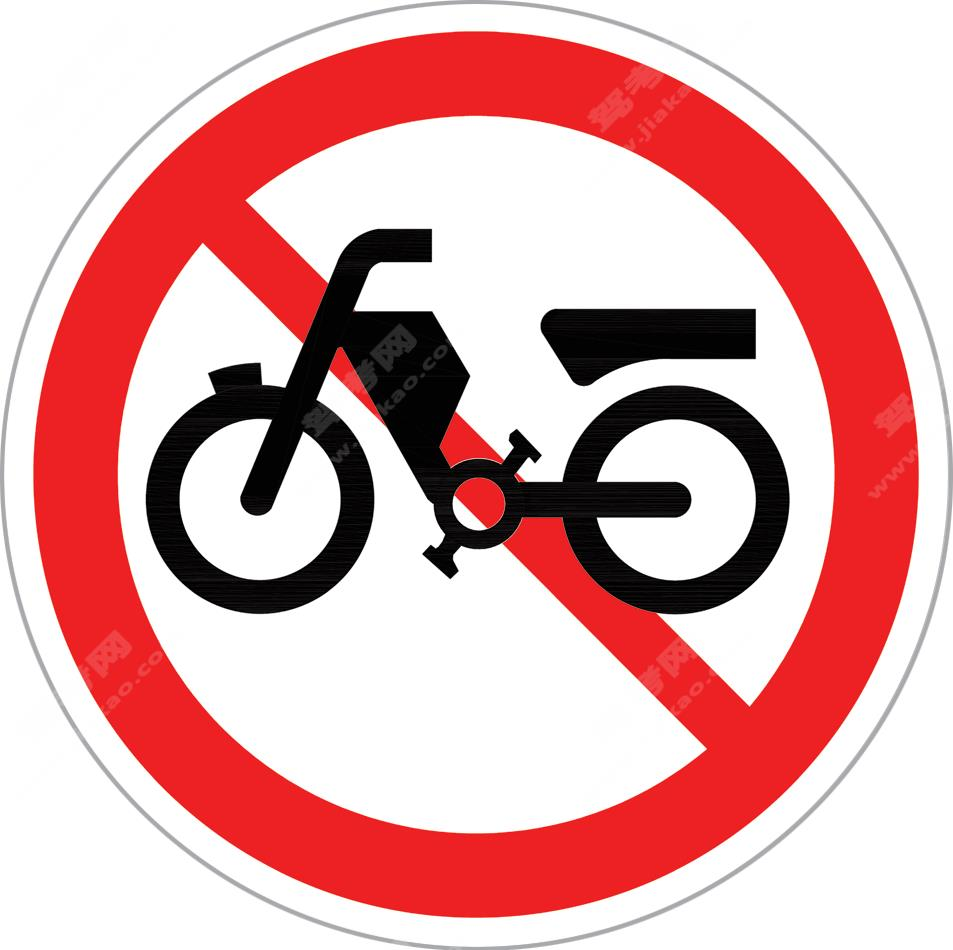禁止电动自行车进入（新）

•禁止大型载客汽车驶入（新）

•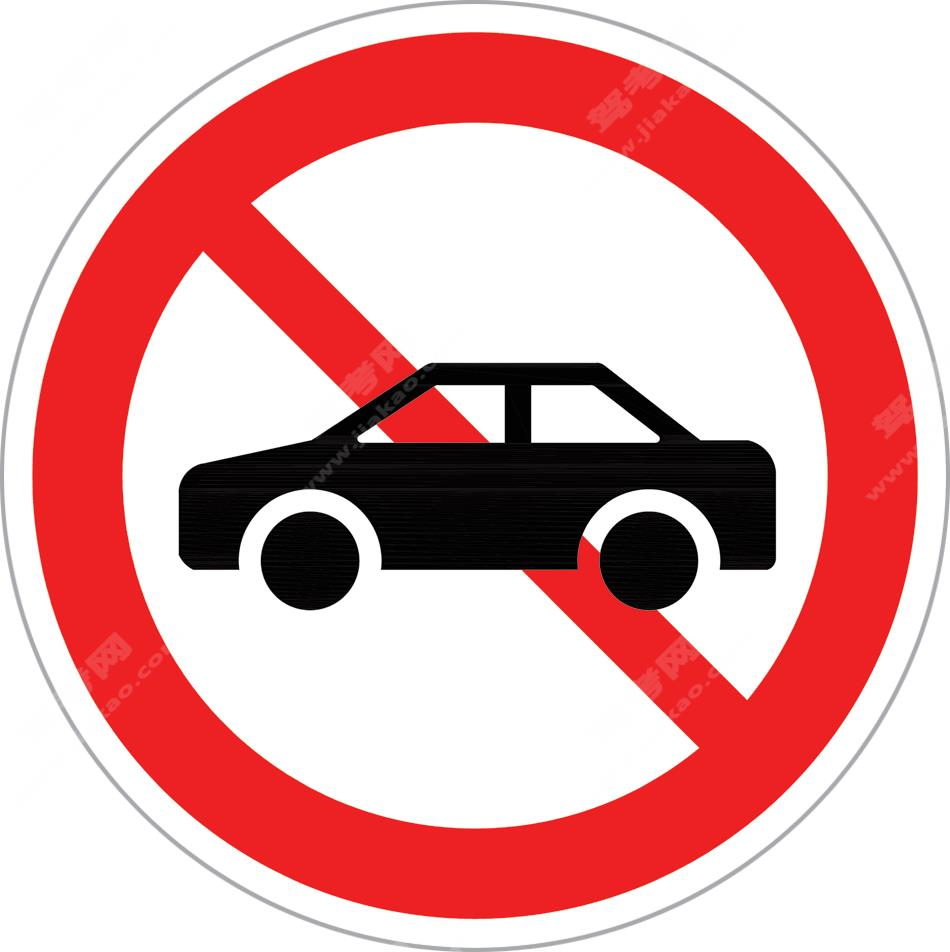禁止小型载客汽车驶入（新）

•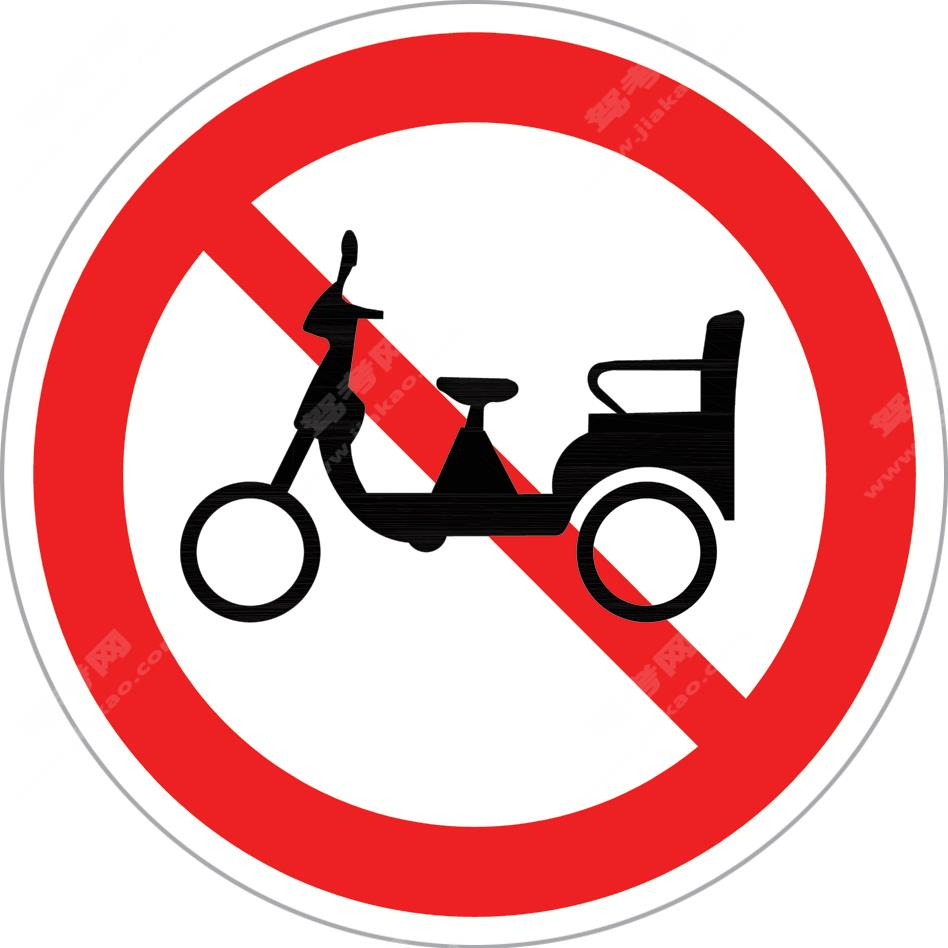禁止三轮车驶入（新）

•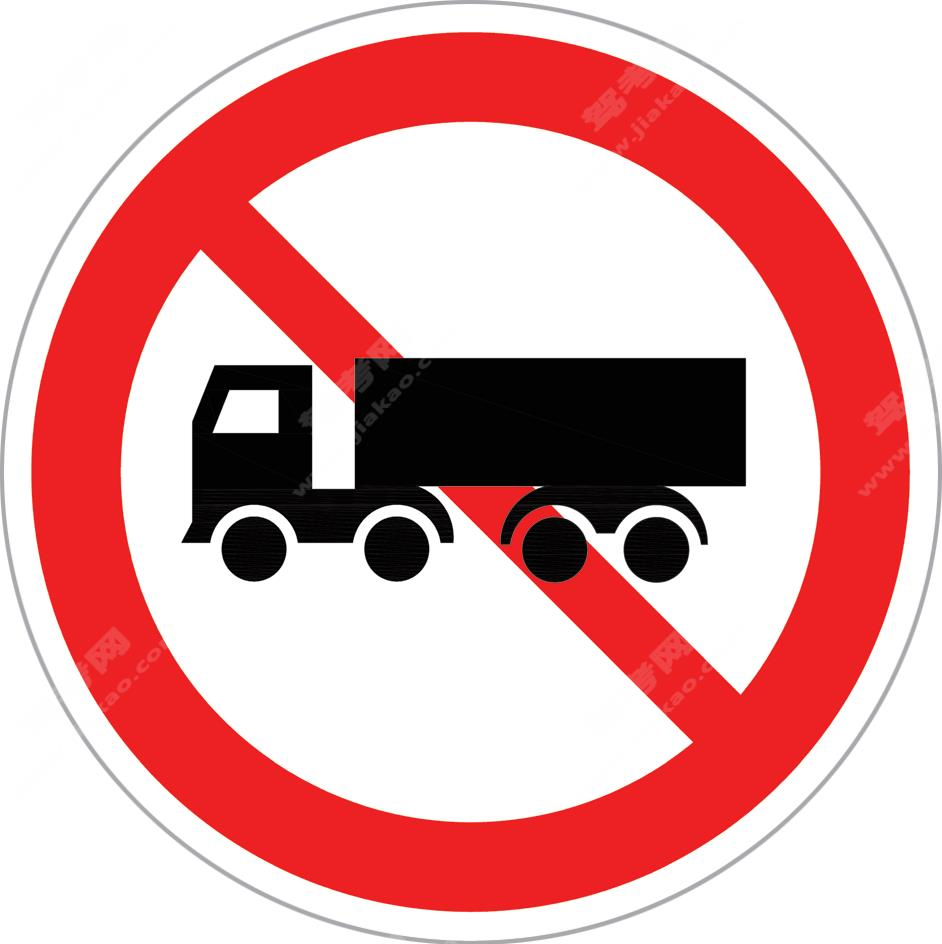禁止挂车、半挂车驶入（新）

•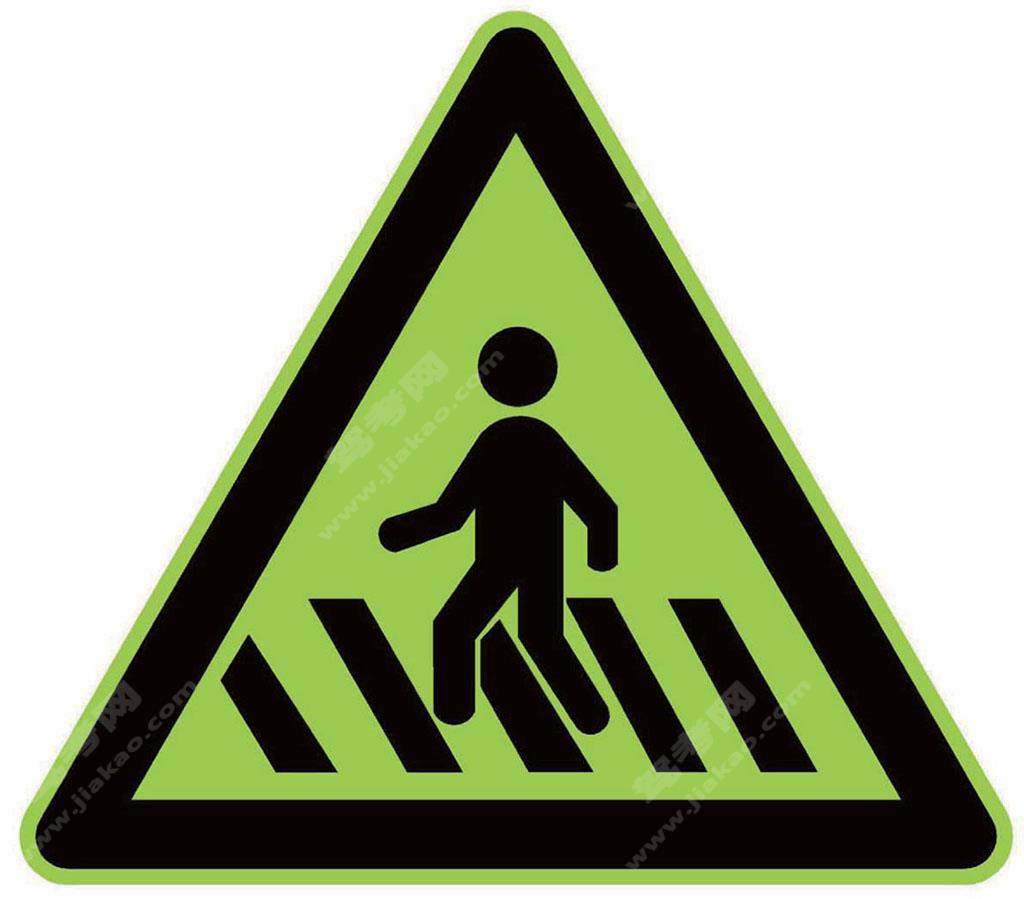注意行人（新）

•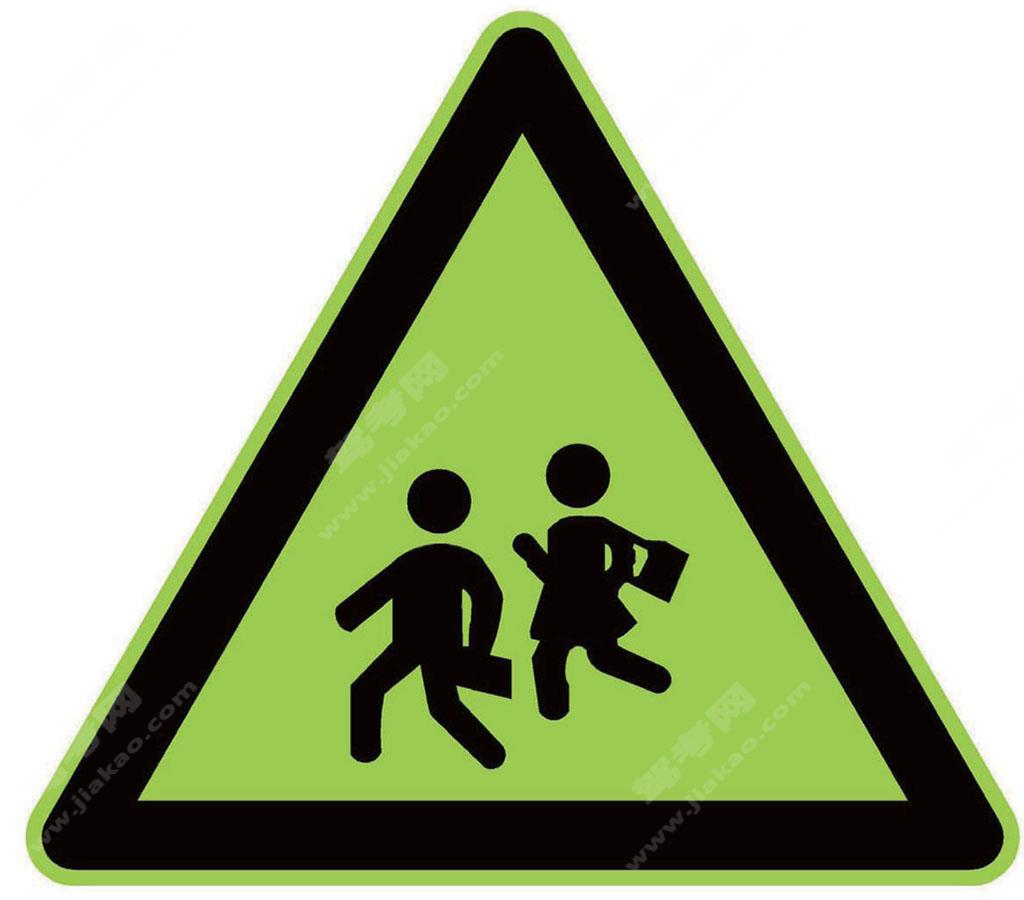注意儿童（新）

•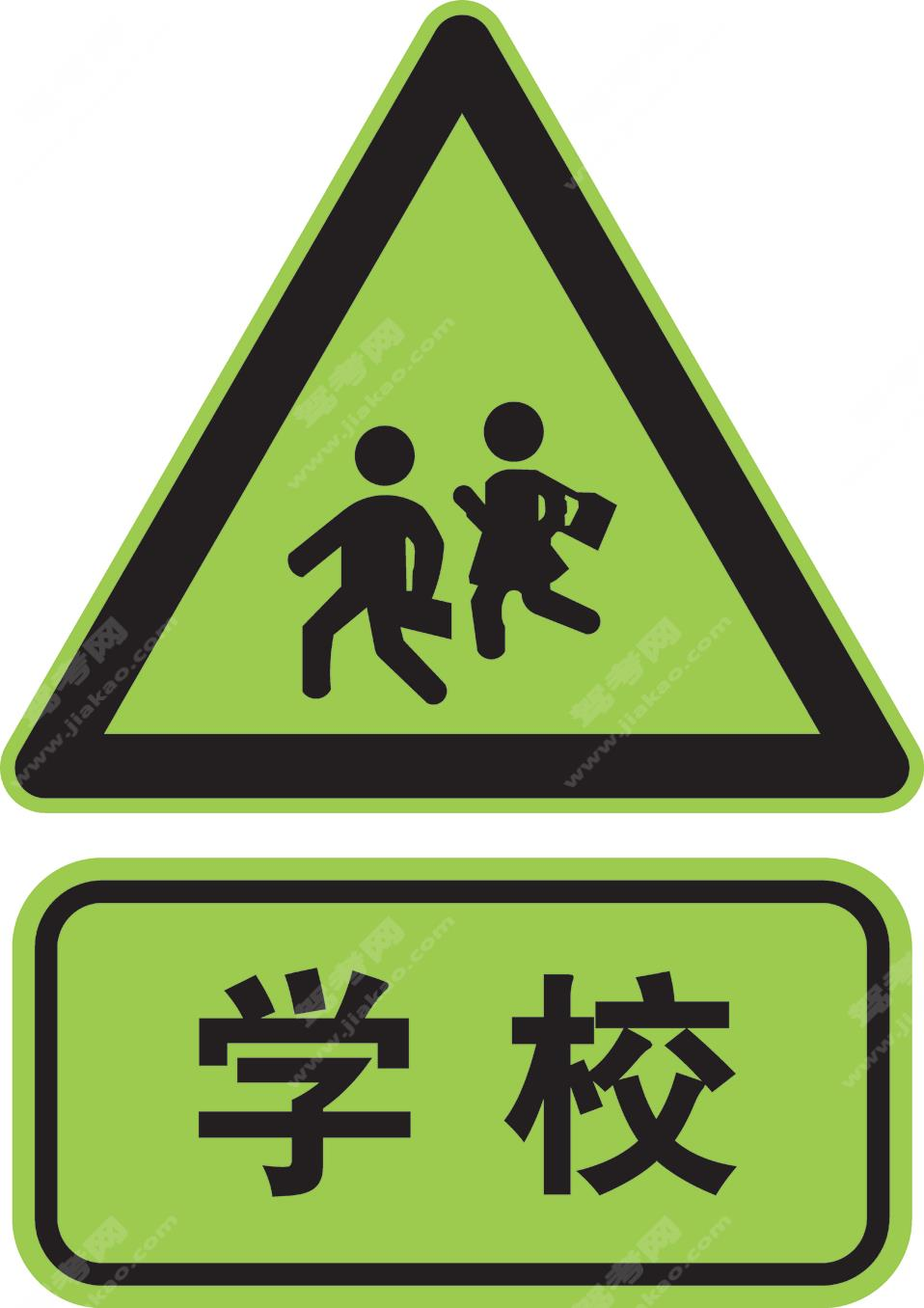注意儿童标志加辅助标志（新）

•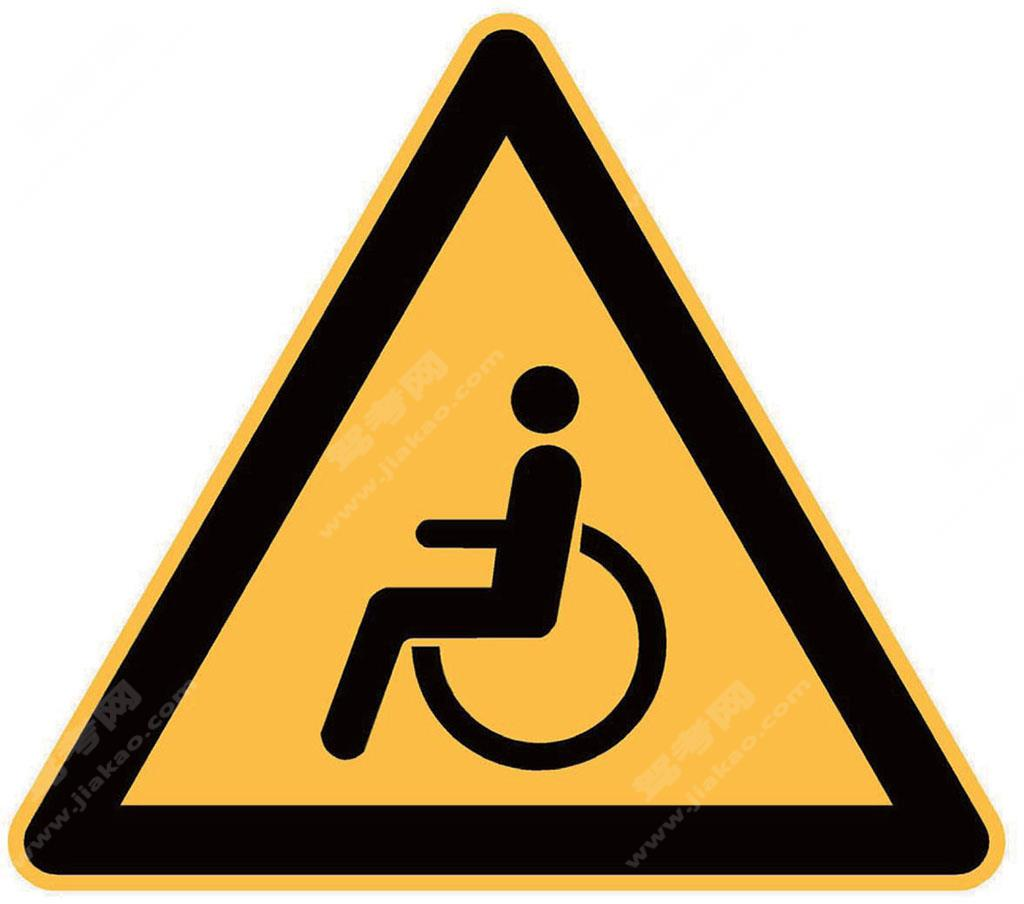注意残疾人（新）

•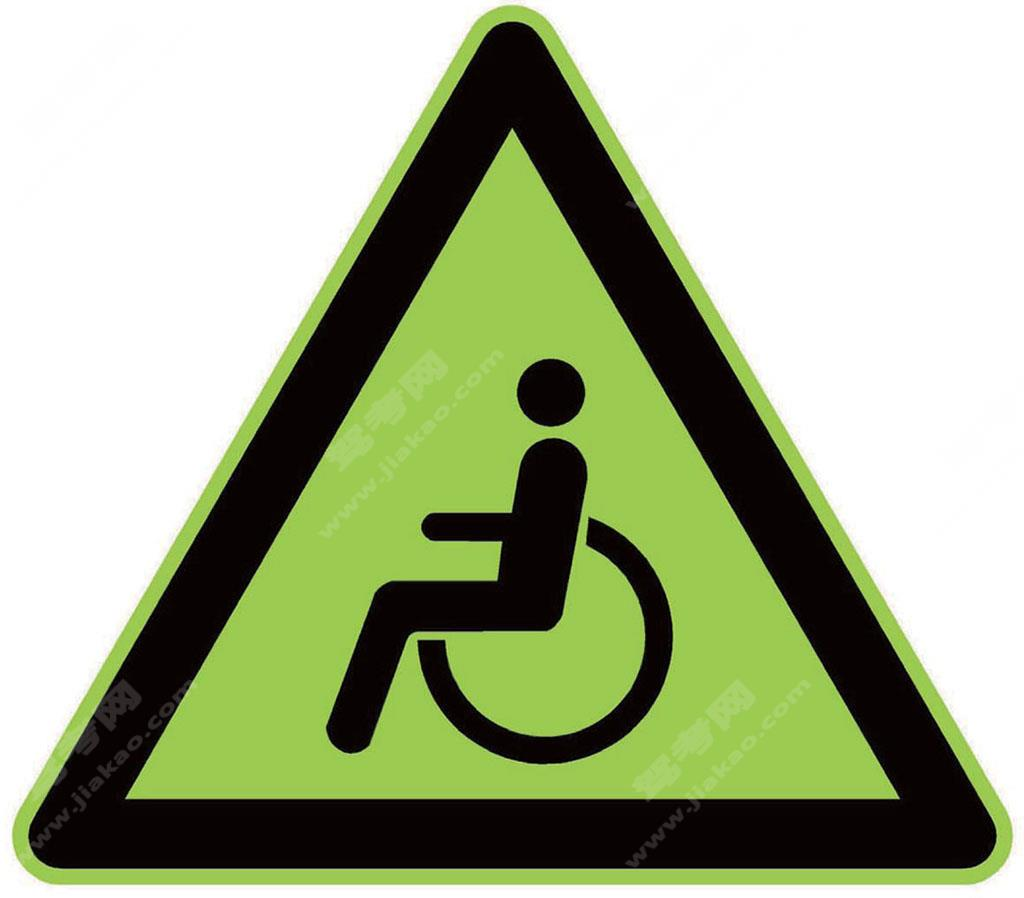注意残疾人（新）

•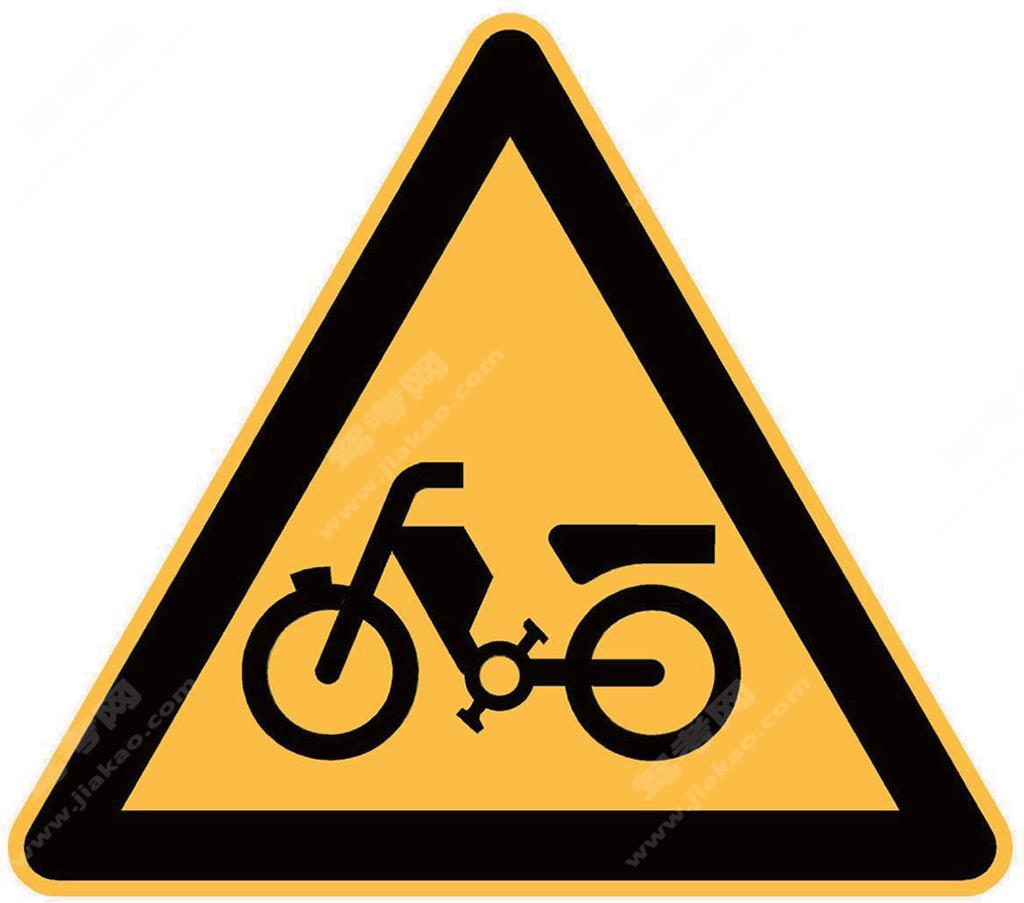注意电动自行车（新）

•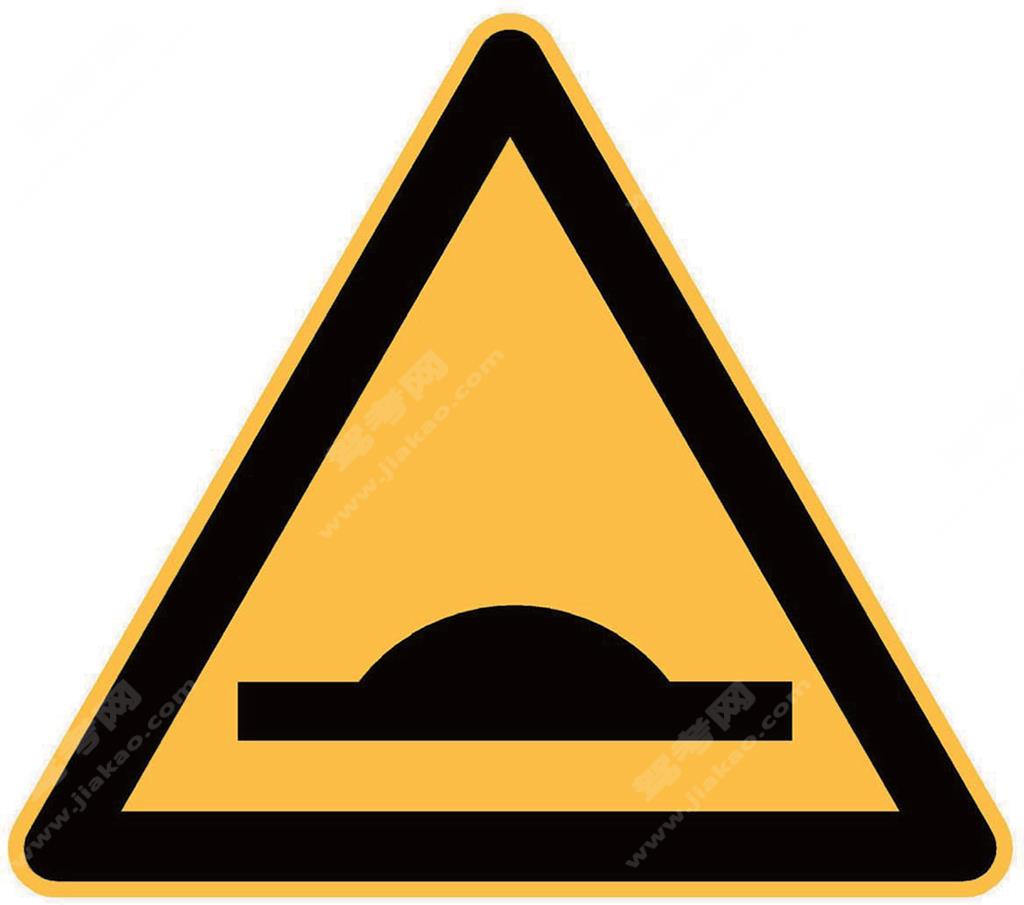减速丘（新）

•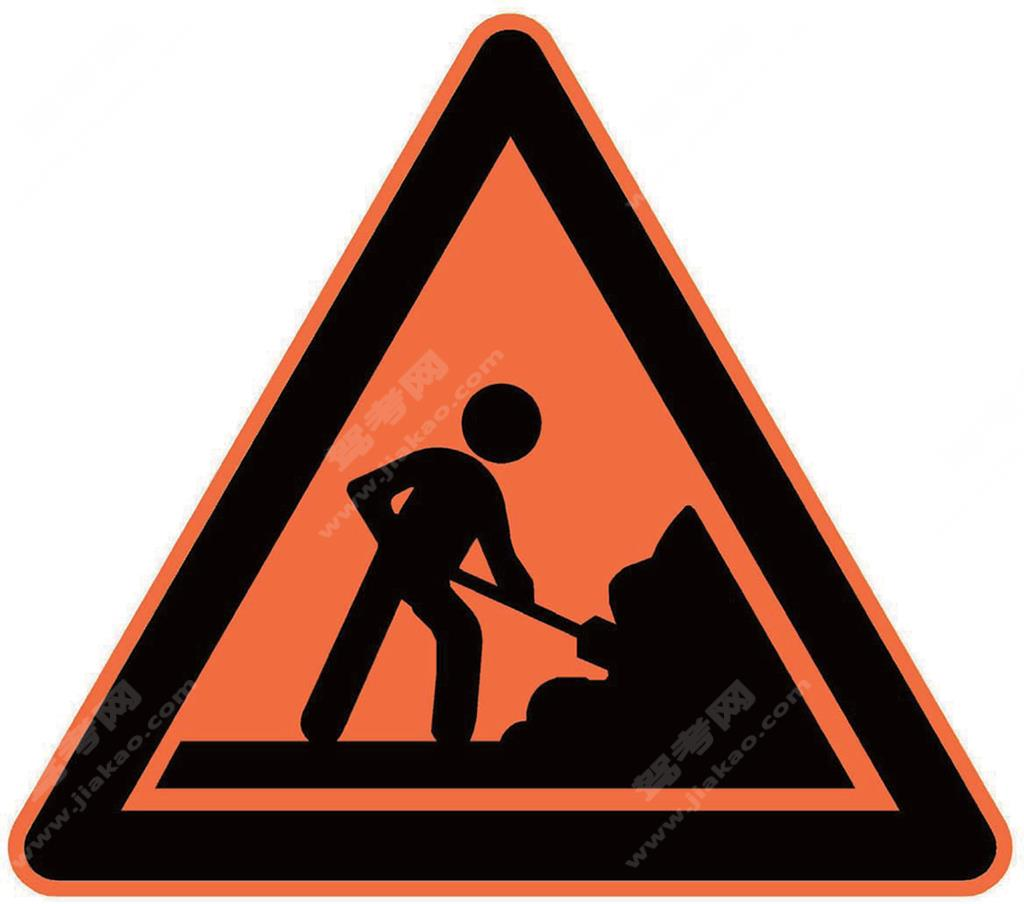施工（新）

•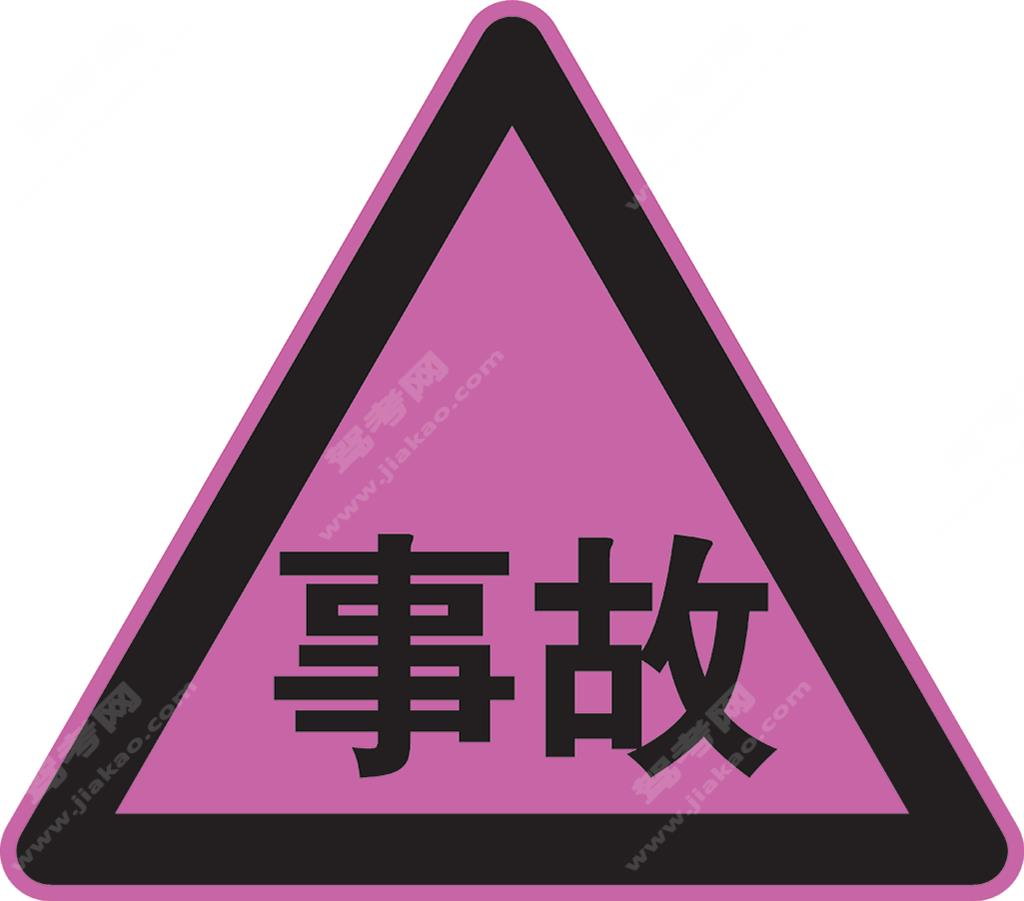交通事故管理（新）

•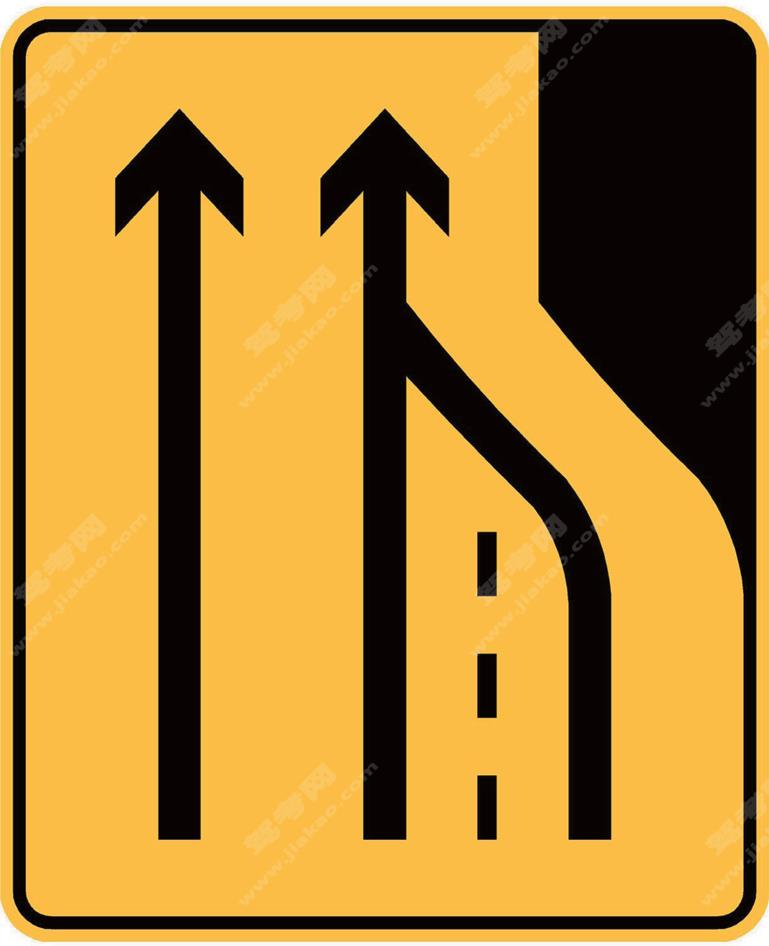车道数变少（新）

•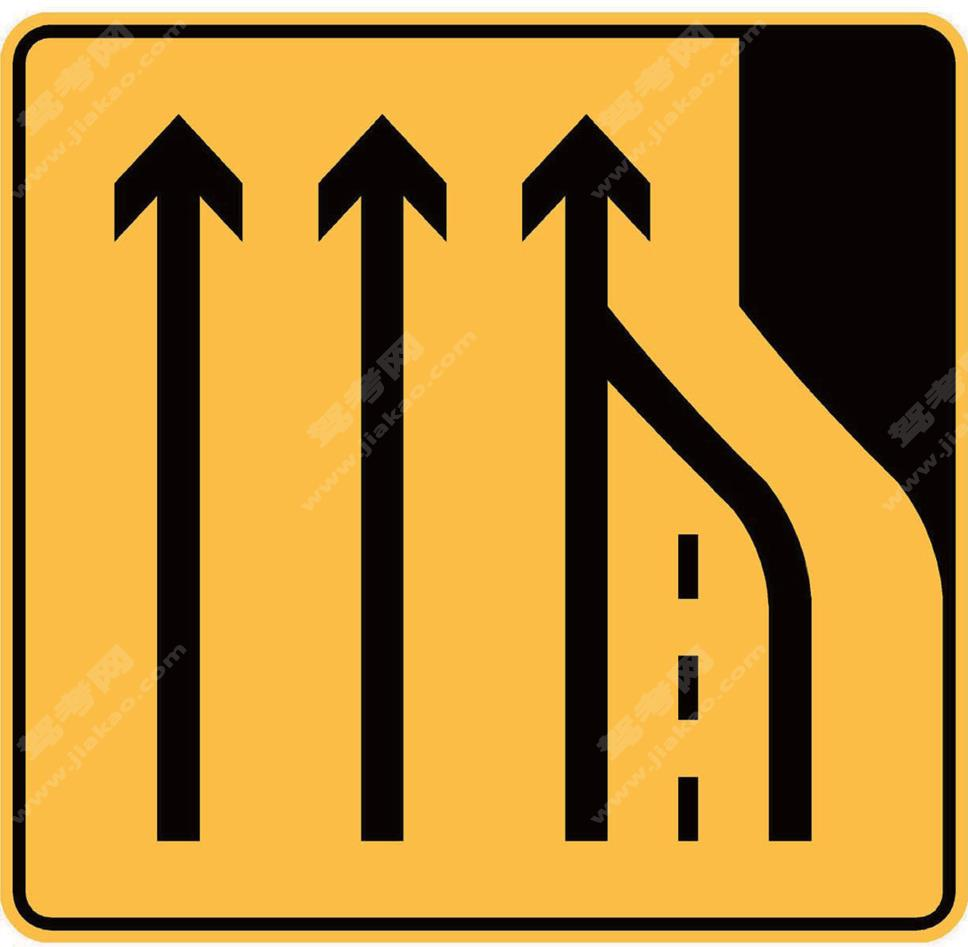车道数变少（新）

•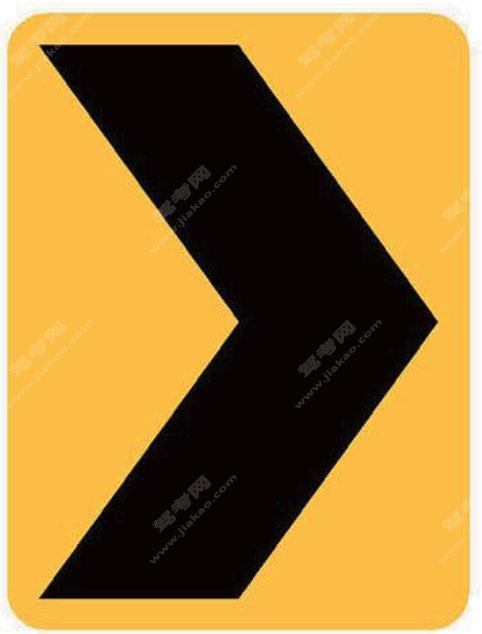线形诱导标（新）

•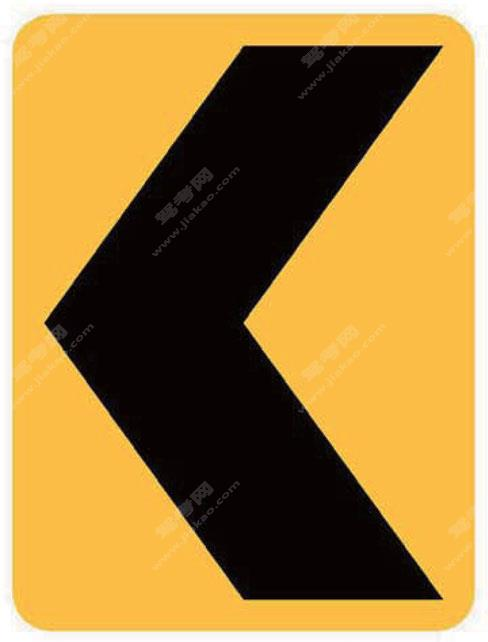线形诱导标（新）

•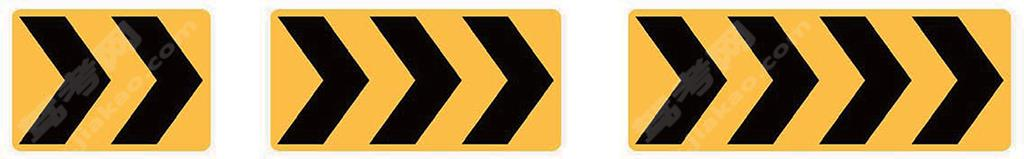线形诱导标（新）

•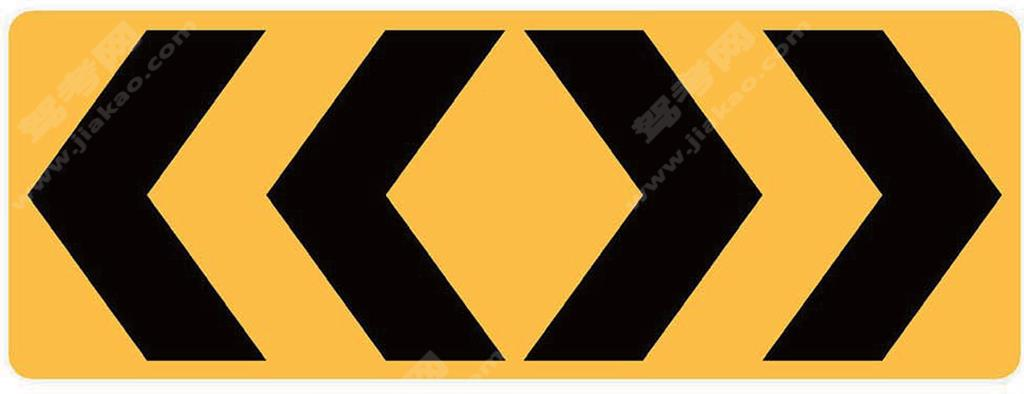线形诱导标（新）

•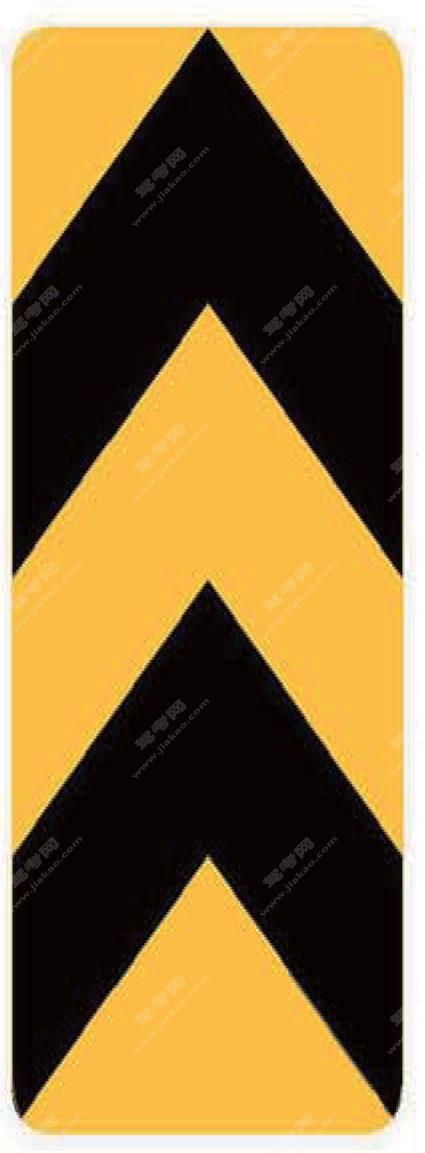两侧通行（新）

•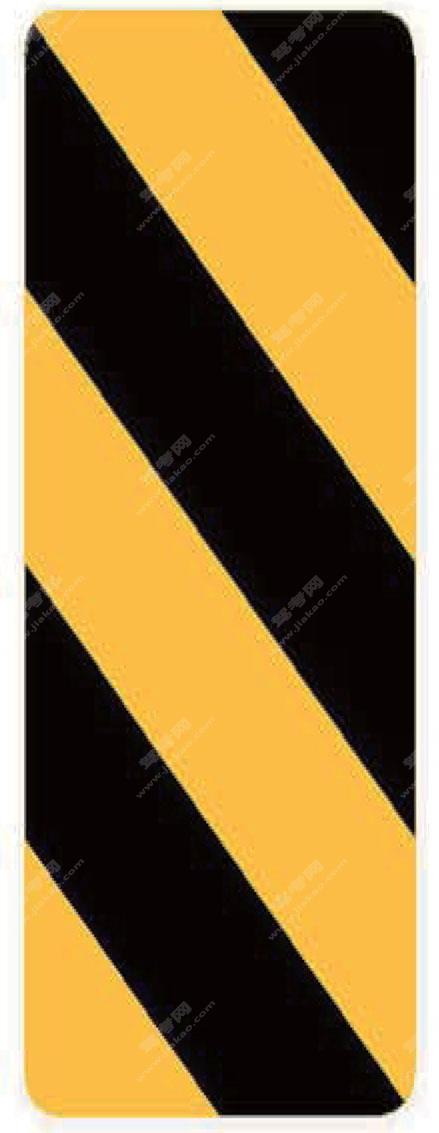右侧通行（新）

•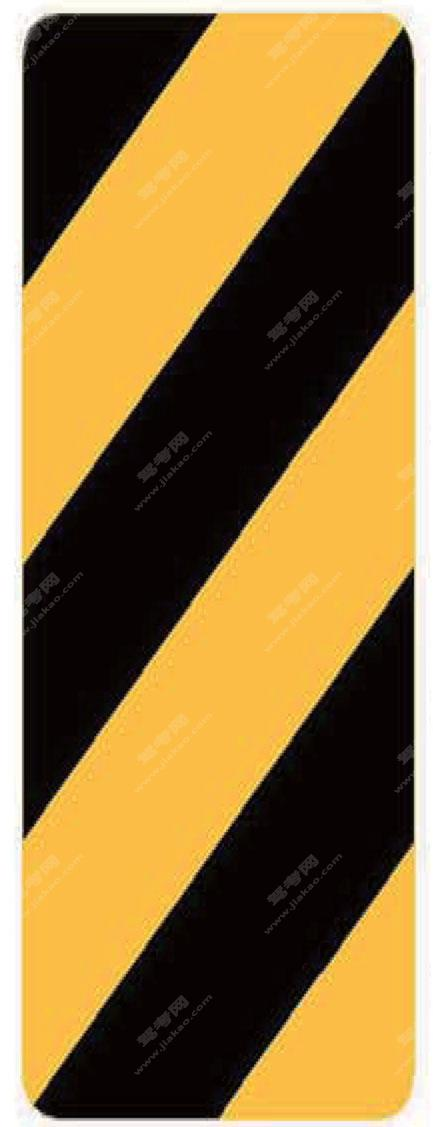左侧通行（新）

•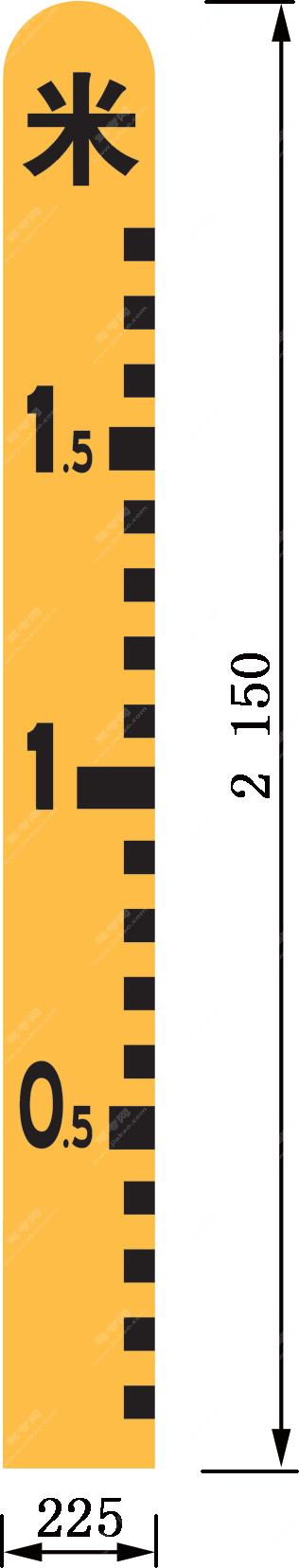注意积水（新）

•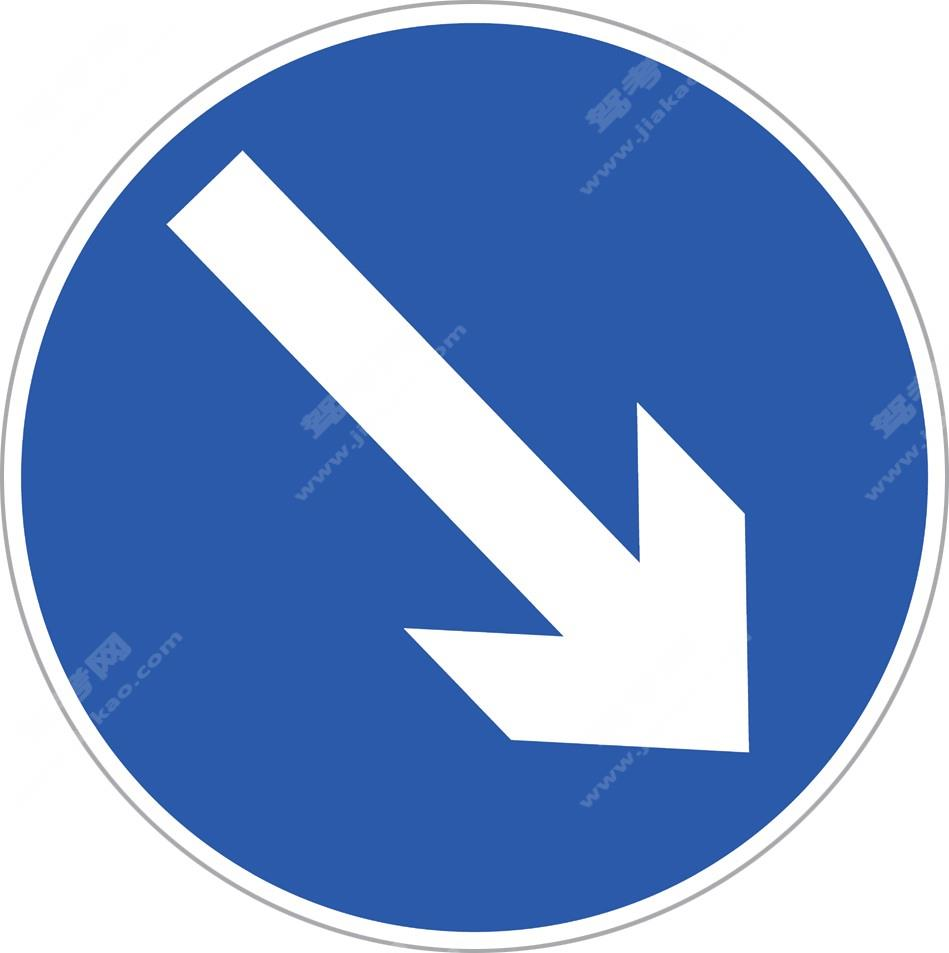分隔带右侧行驶（新）

•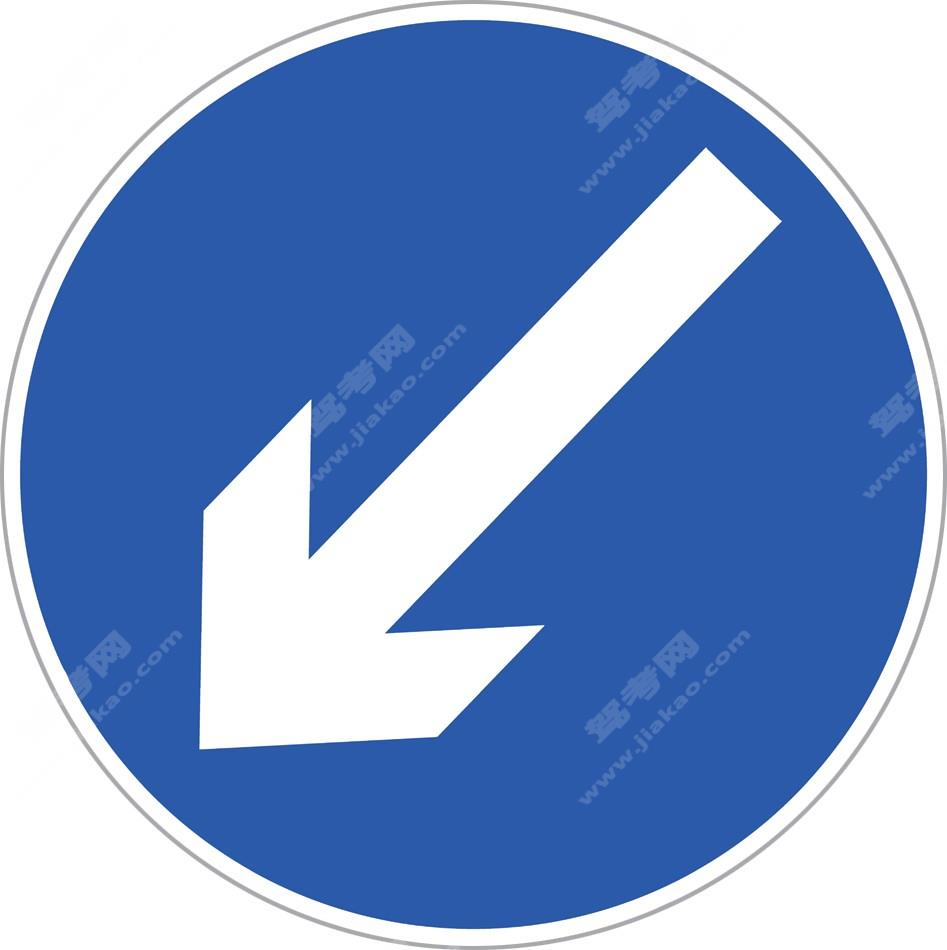分隔带左侧行驶（新）

•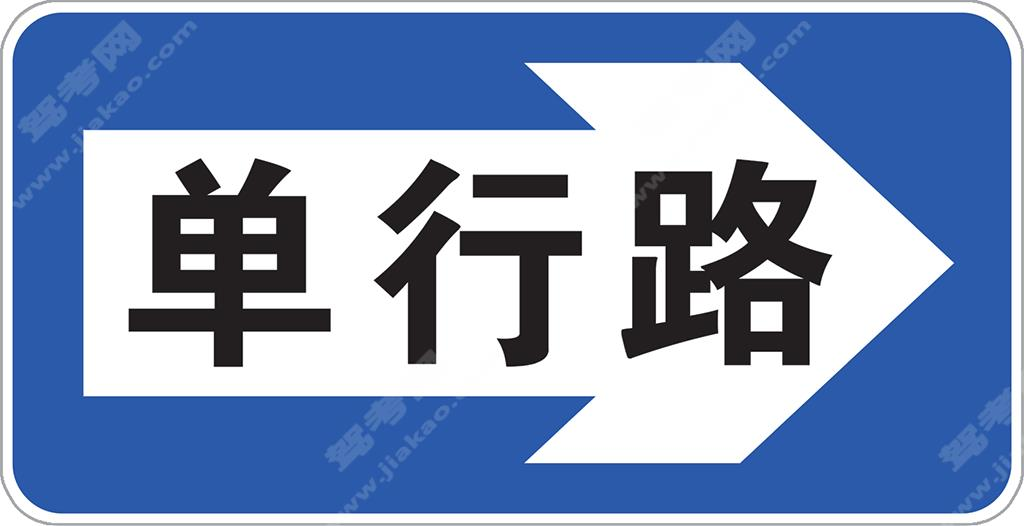单行路（向右）（新）

•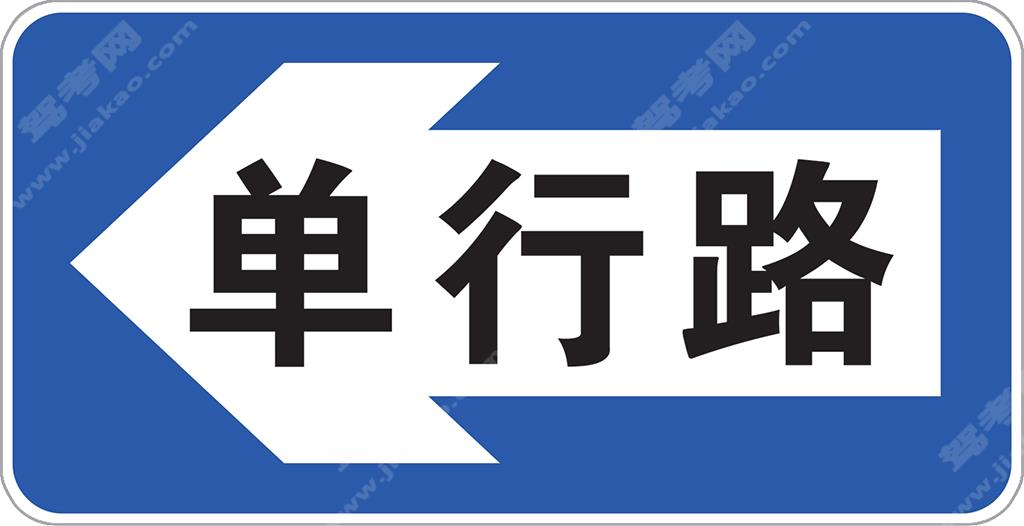单行路（向左）（新）

•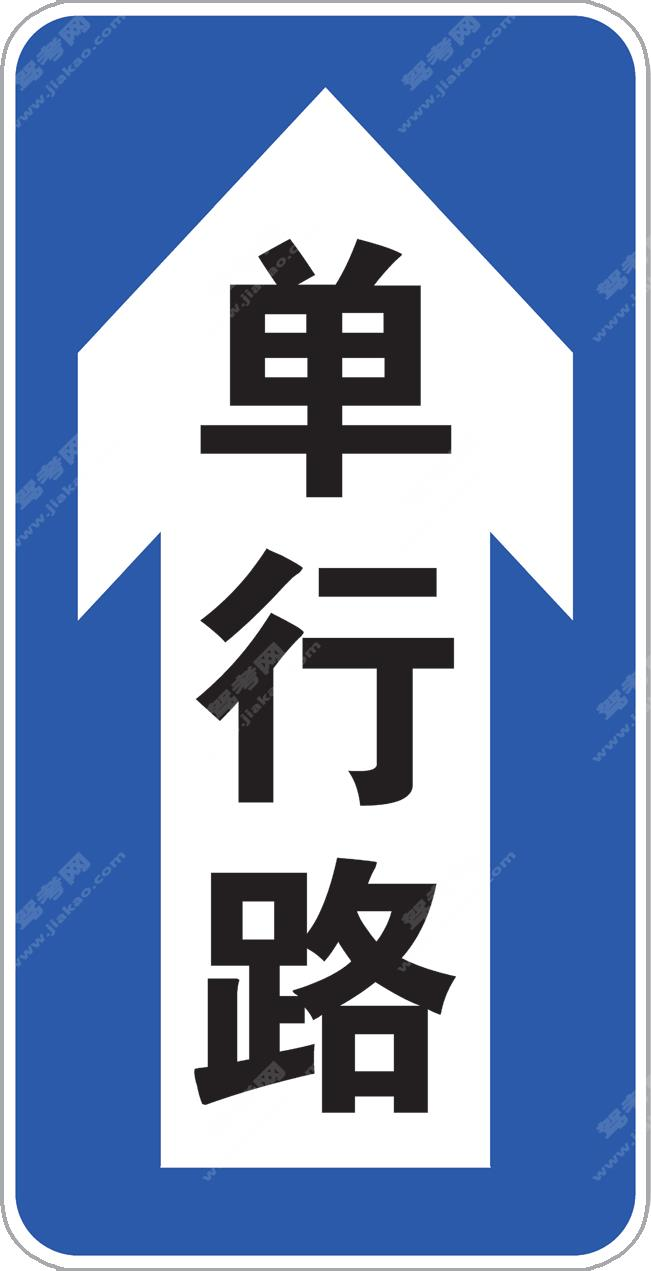单行路（直行）（新）

•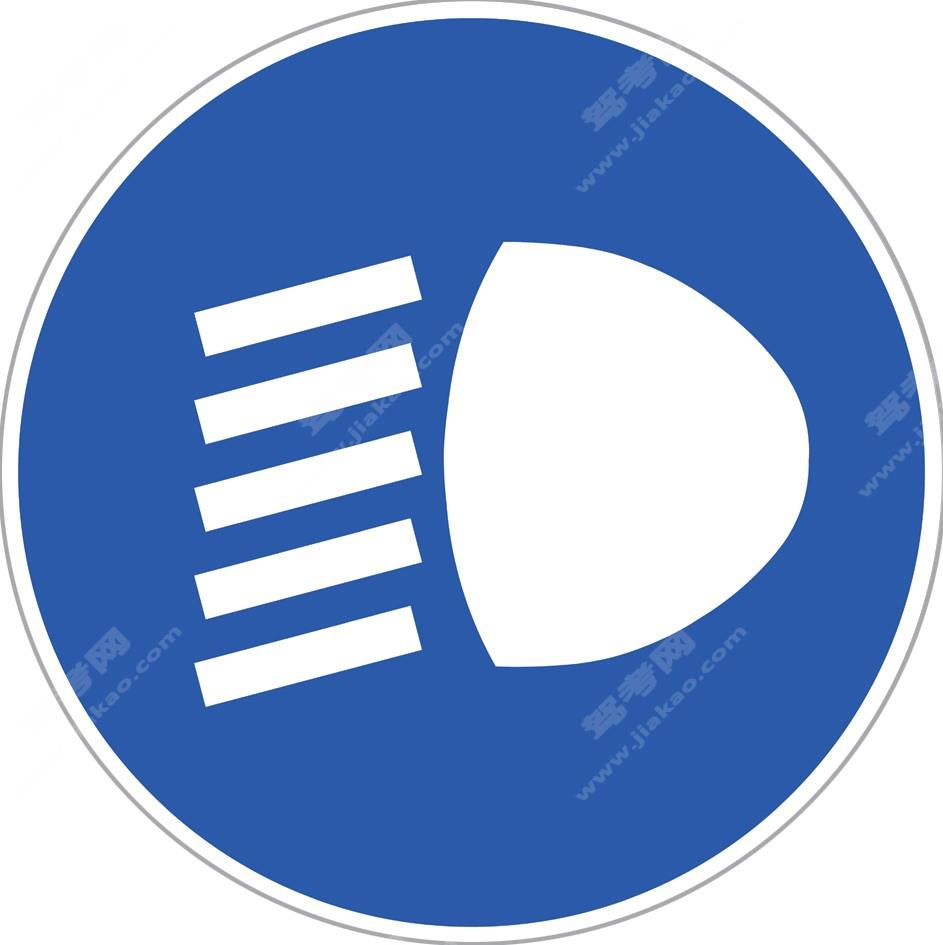开车灯（新）

•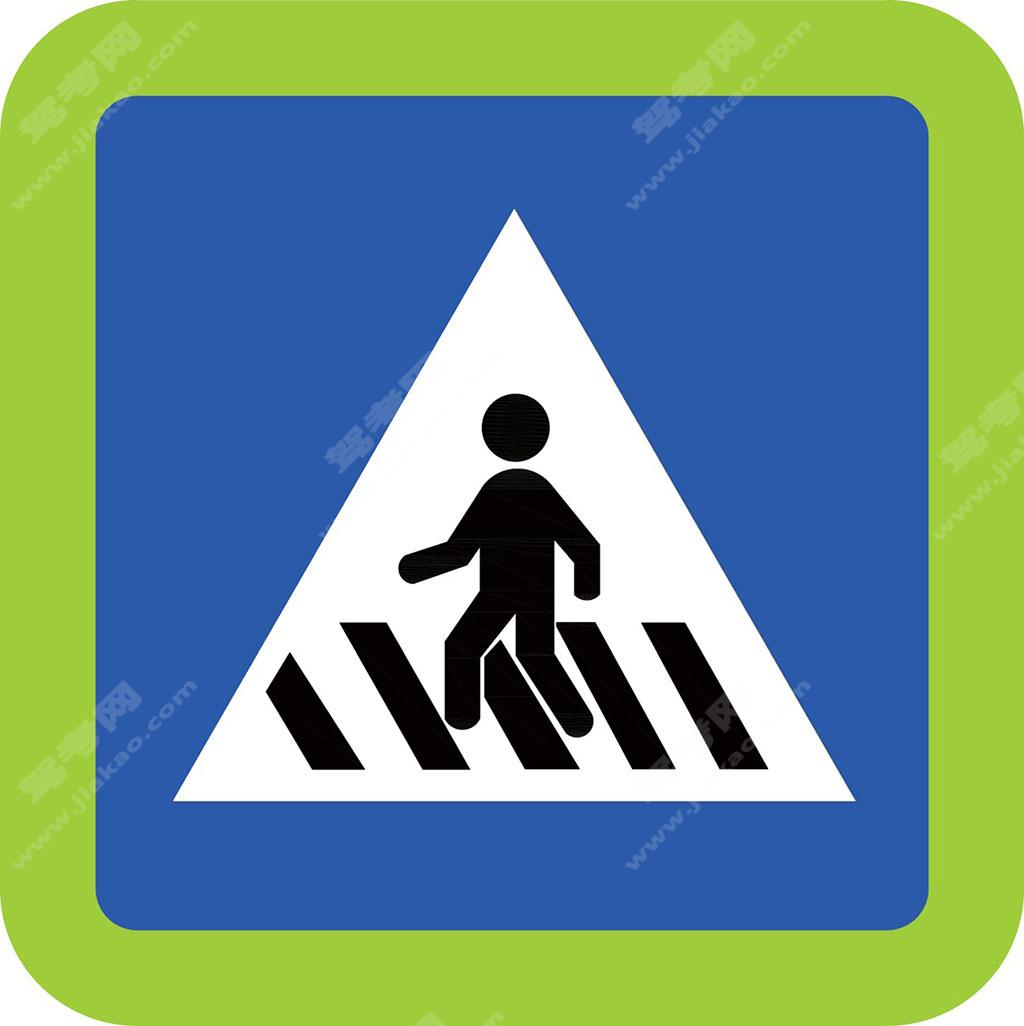人行横道（新）

•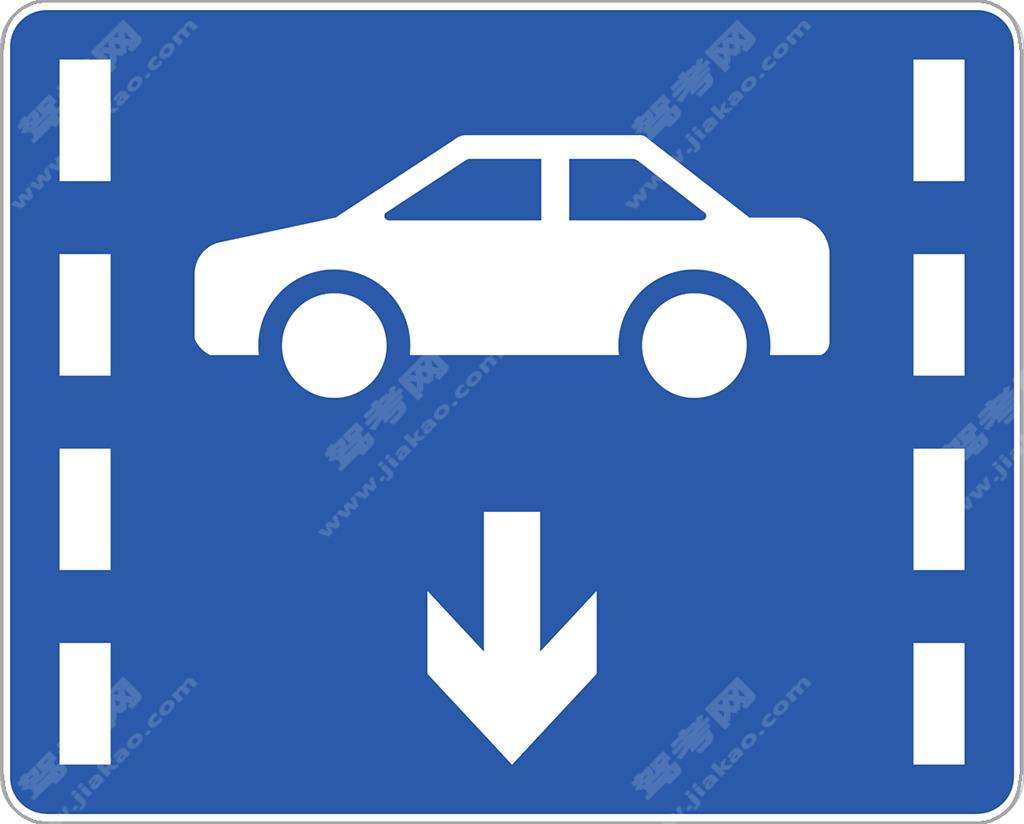小型客车车道

•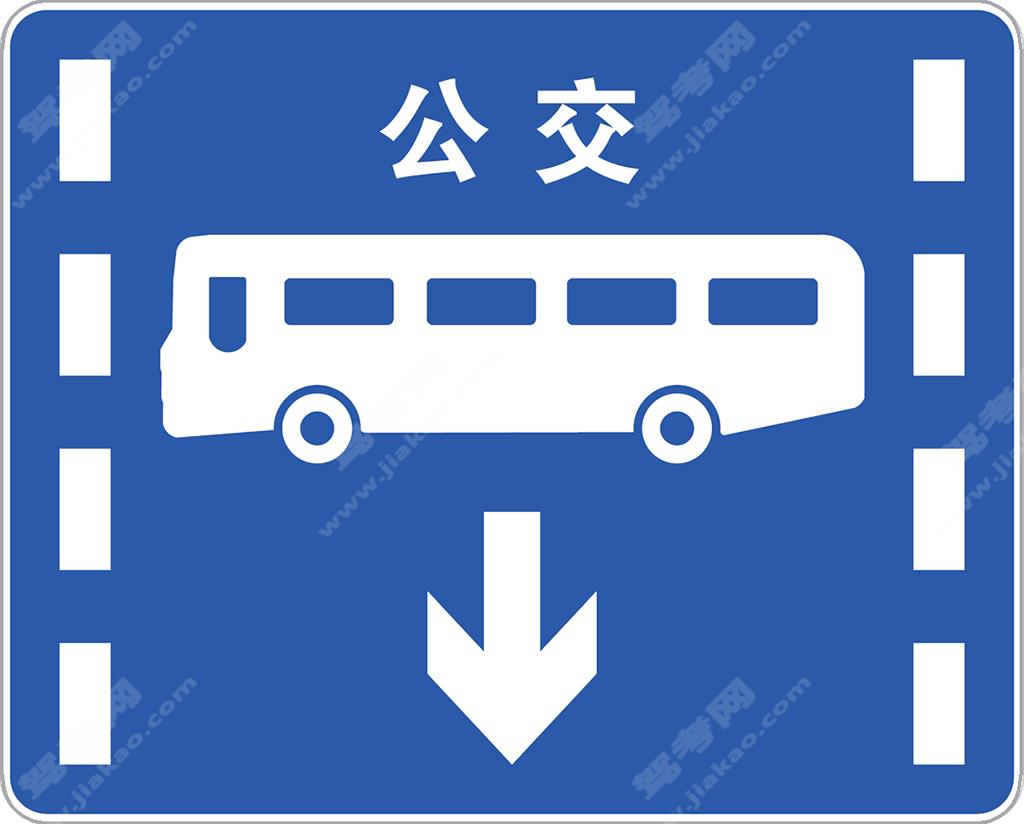公交专用车道（新）

•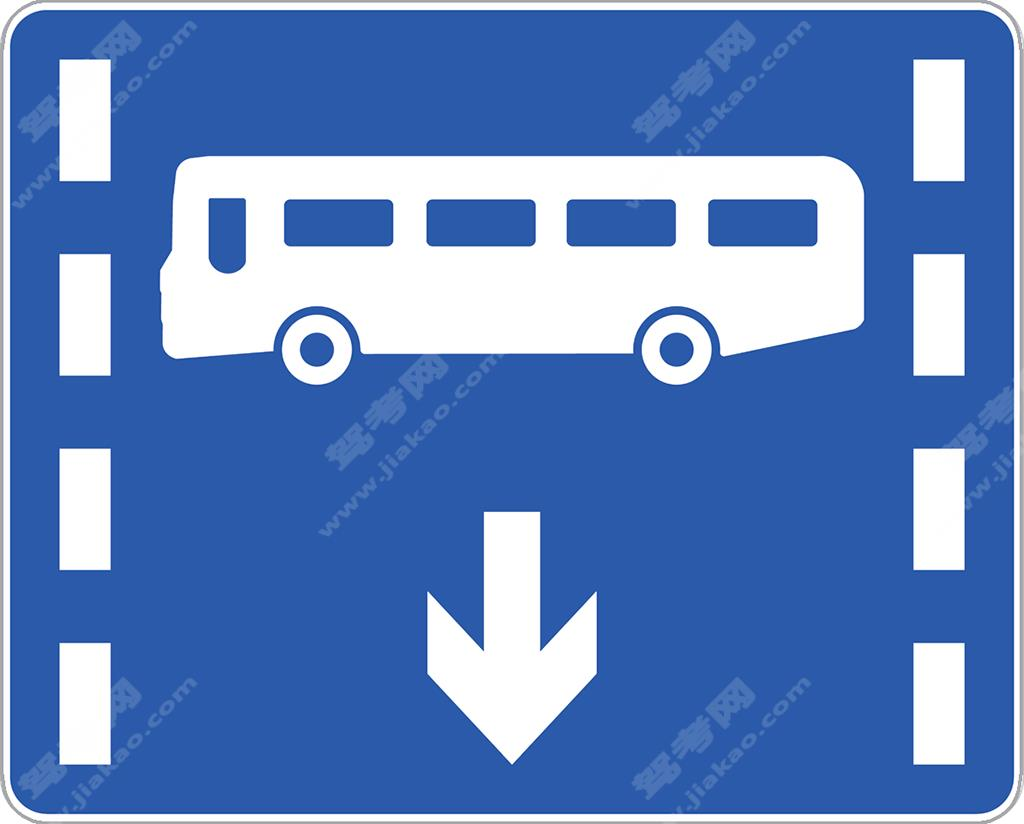公交专用车道（新）

•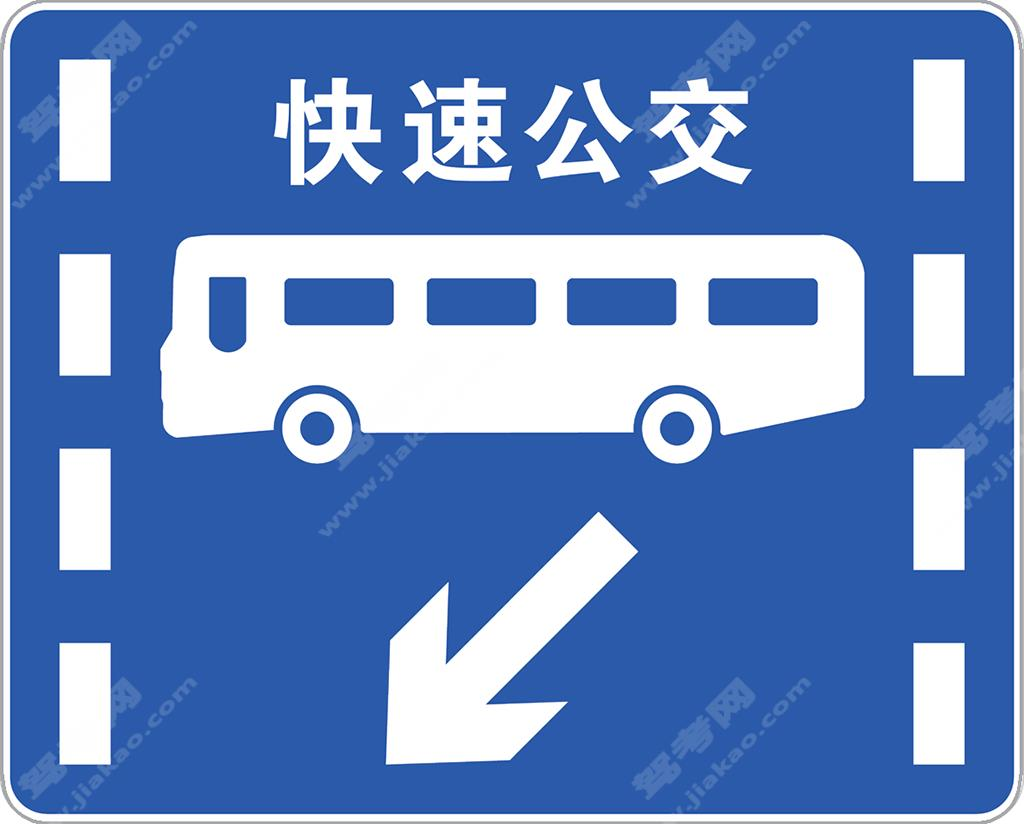快速公交系统专用车道（新）

•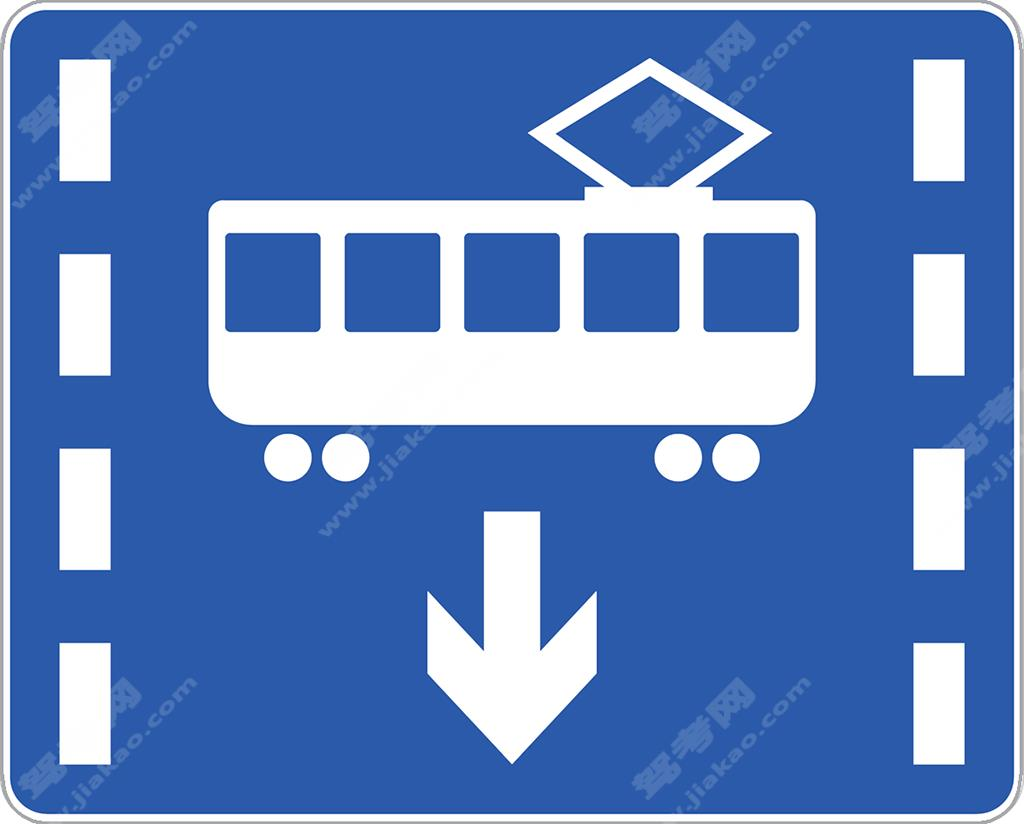有轨电车专用车道（新）

•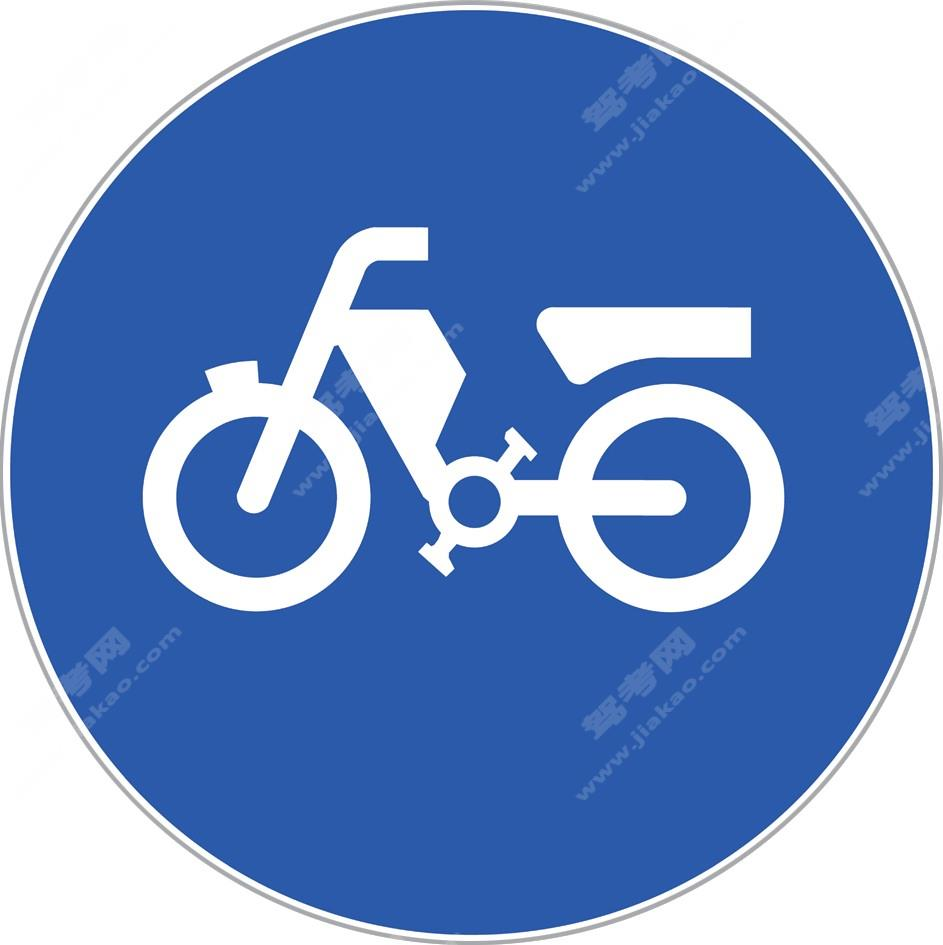电动自行车行驶（新）

•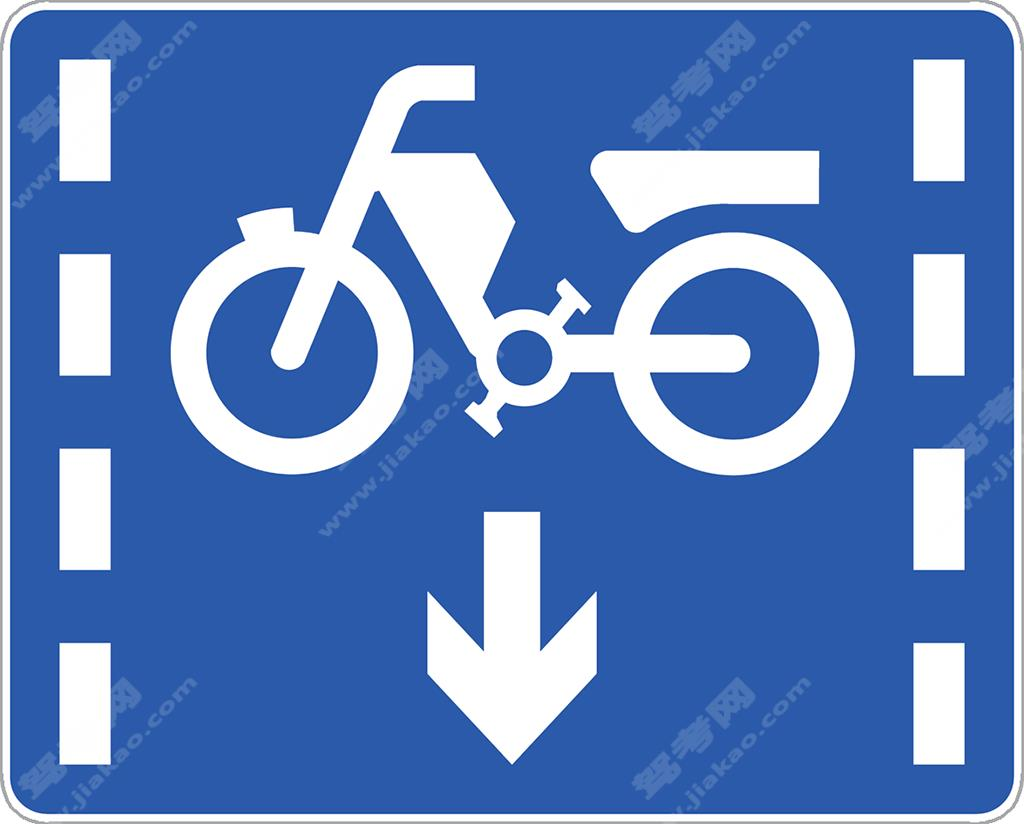电动自行车车道（新）

•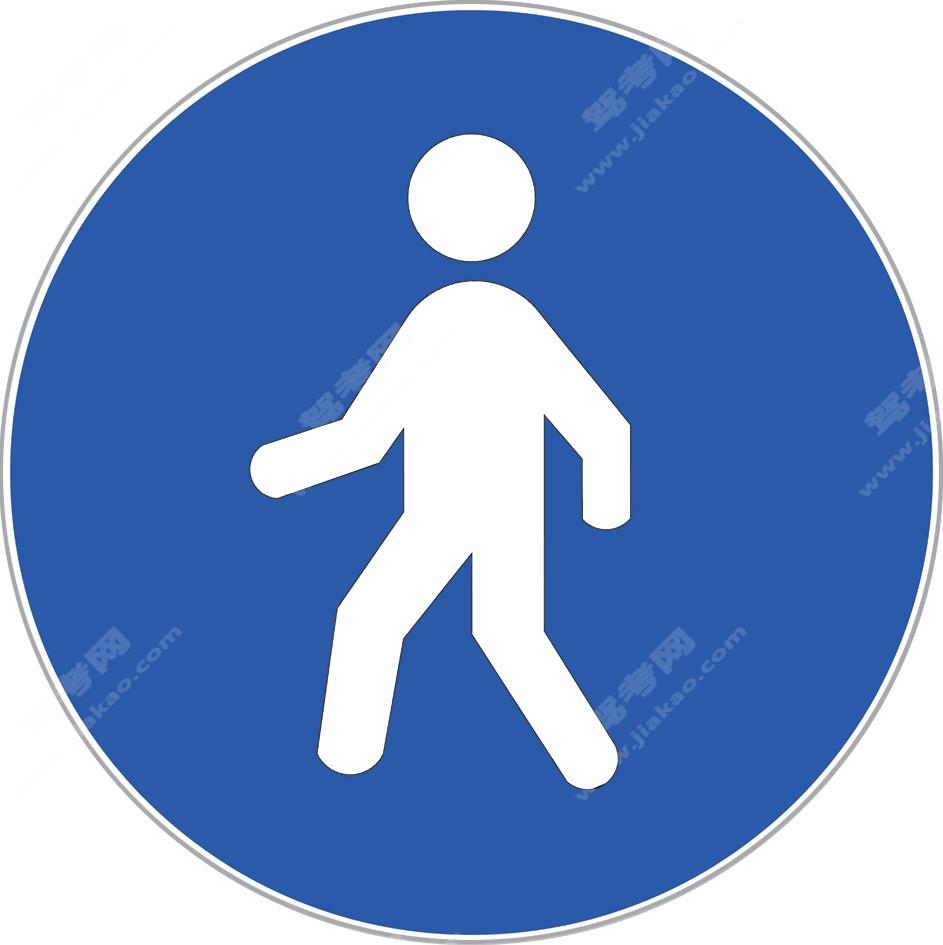行人（新）

•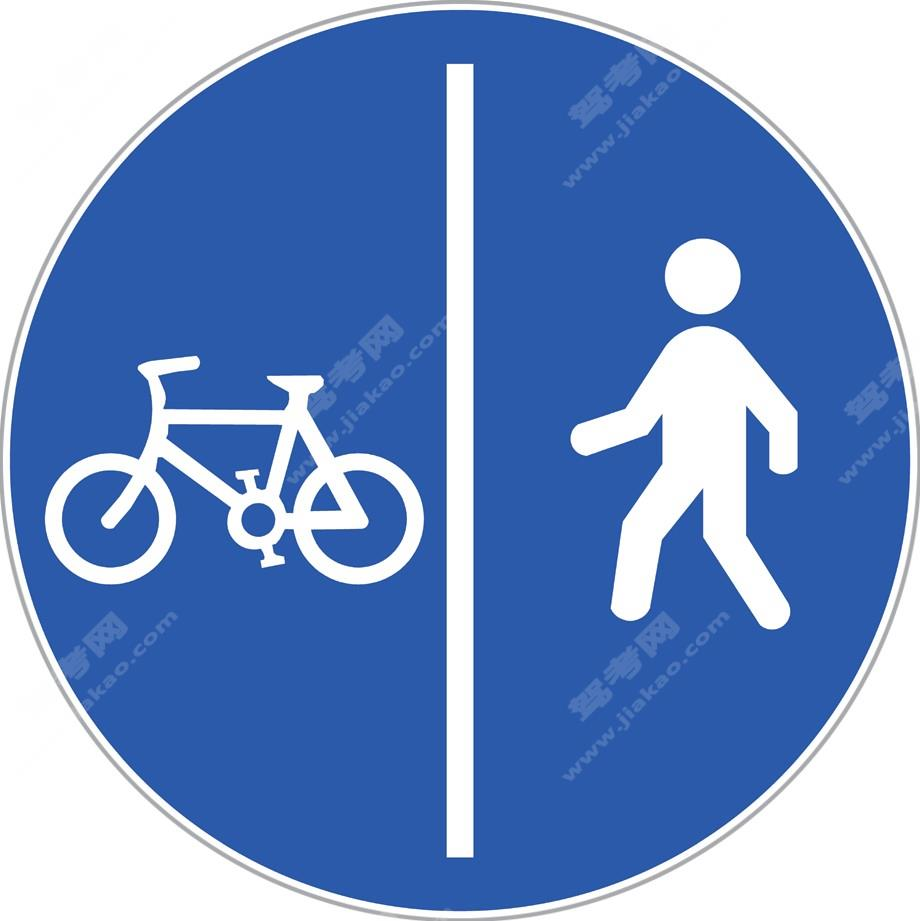非机动车与行人分开空间通行（新）

•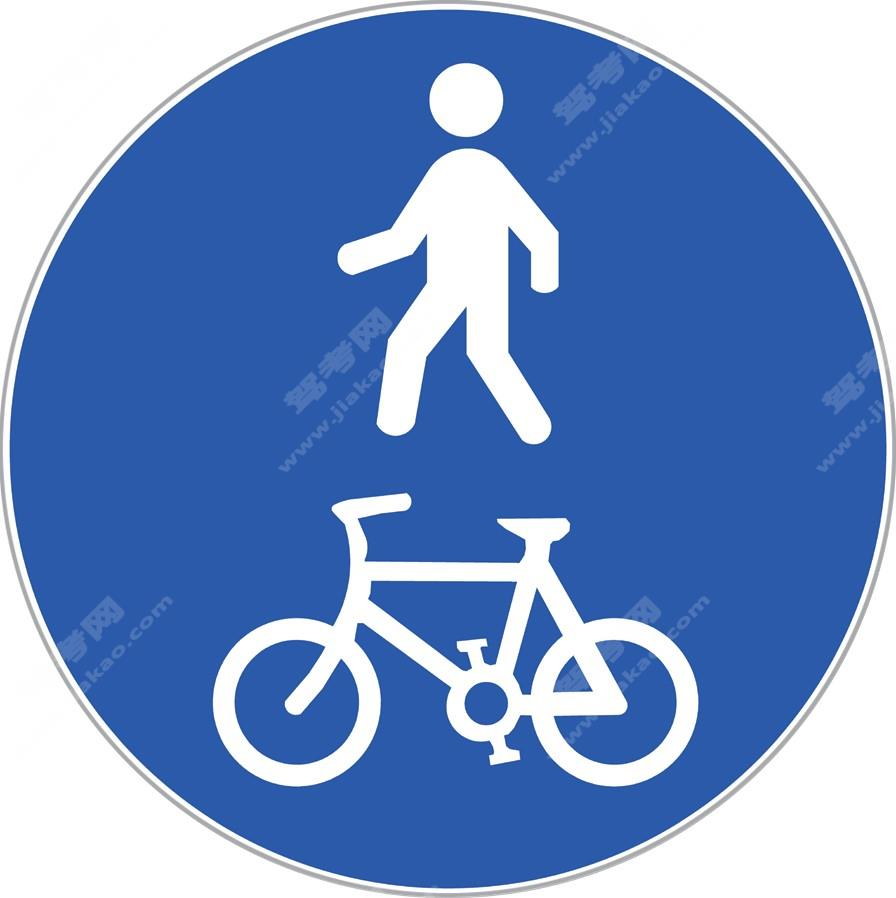非机动车与行人共享空间通行（新）

•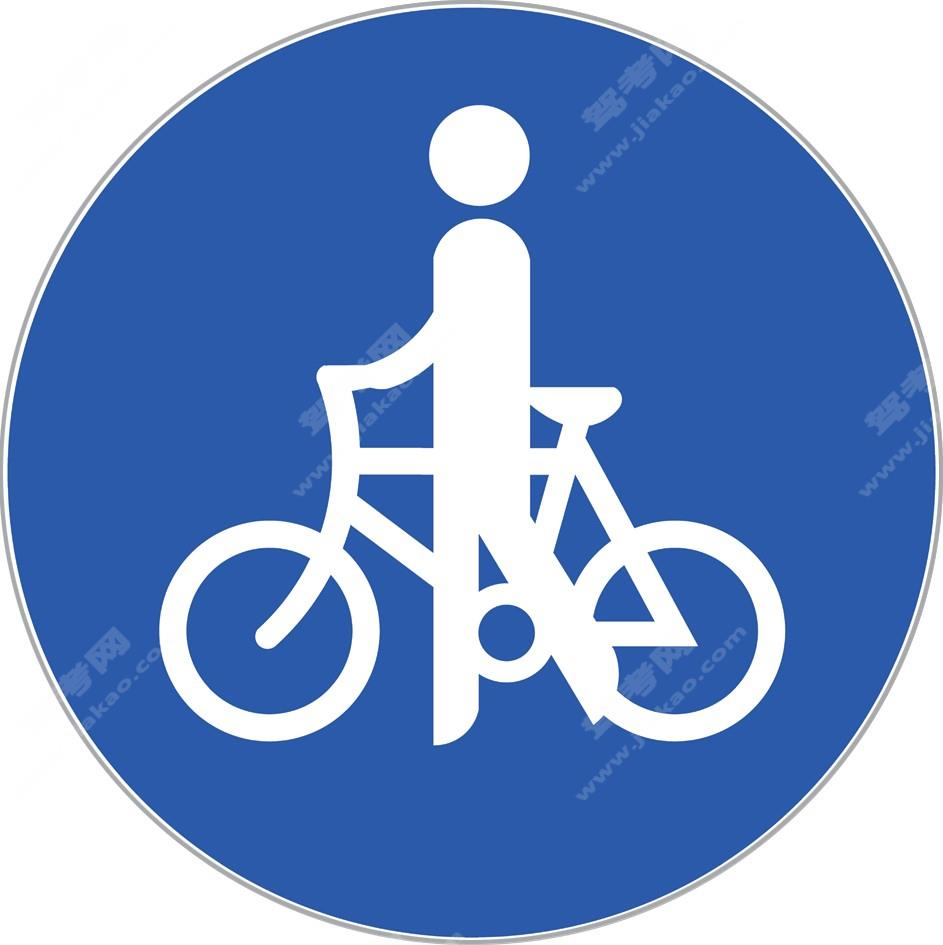非机动车推行（新）

•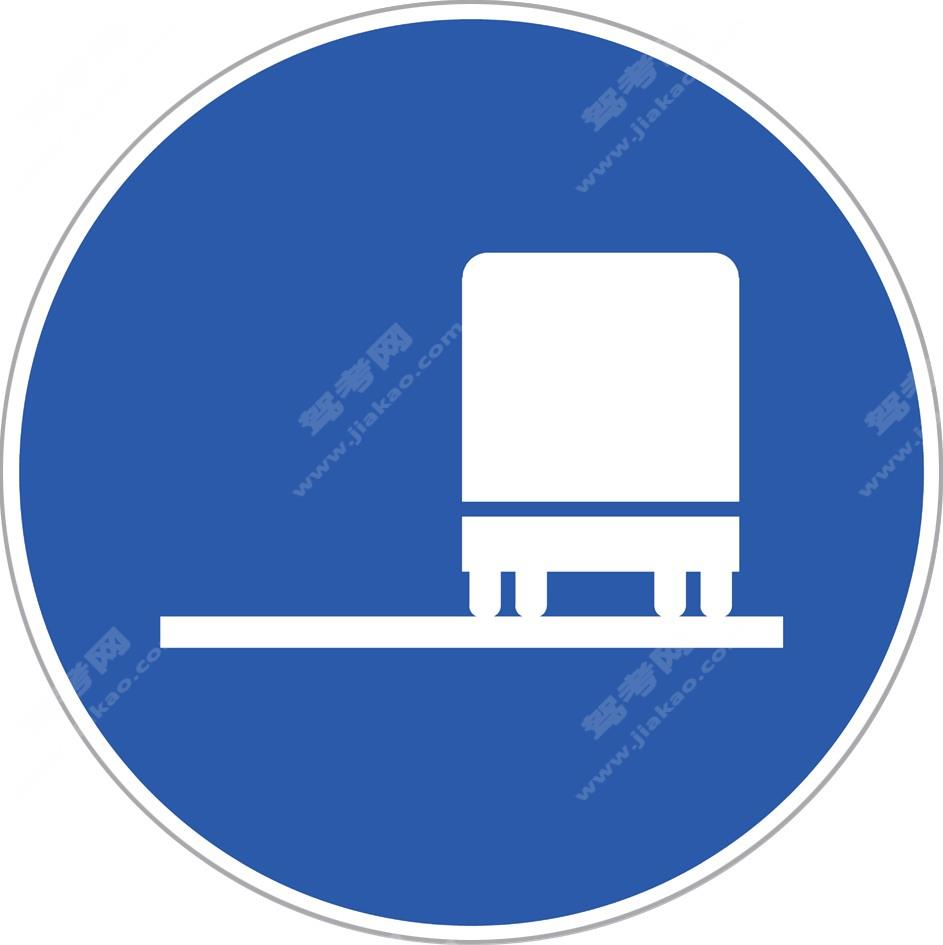靠右侧车道行驶（新）

•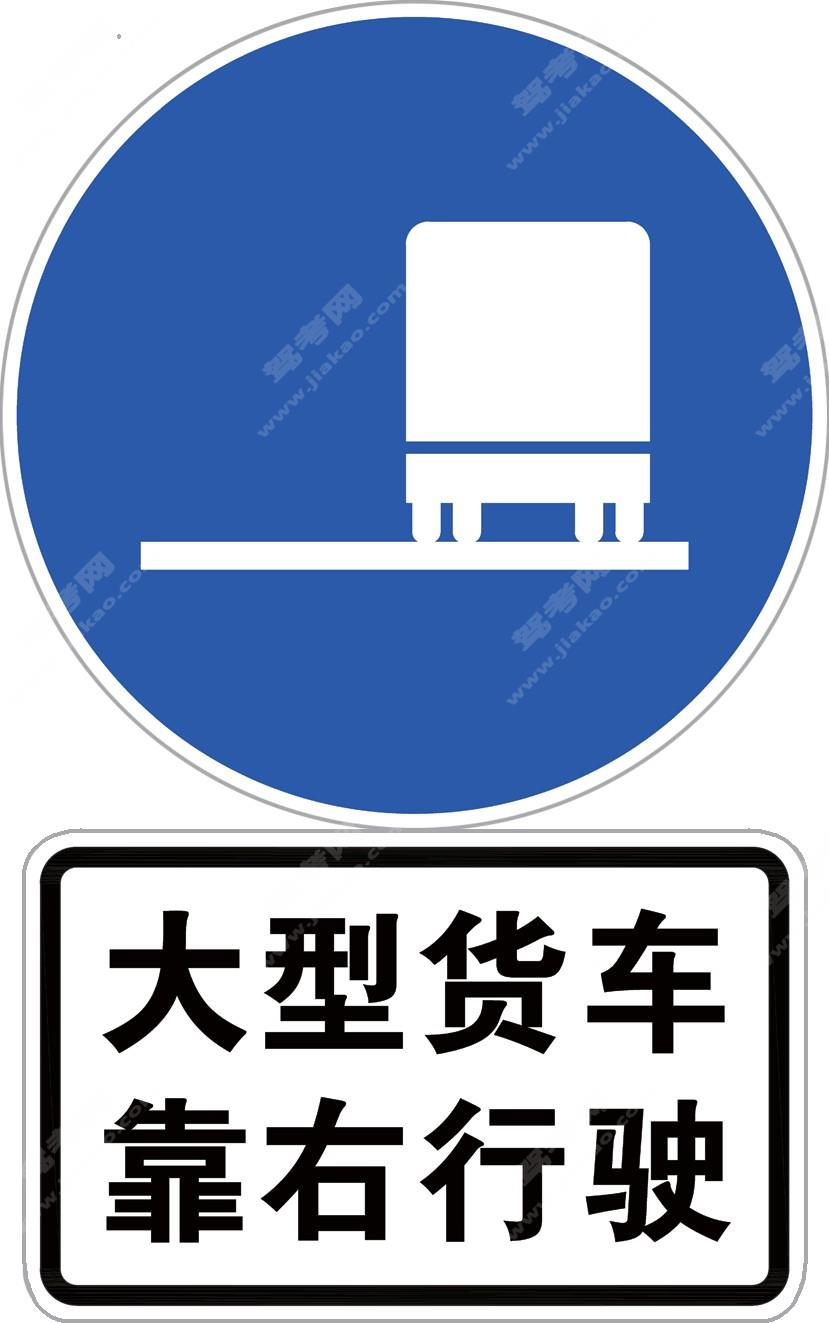靠右侧车道行驶标志加辅助标志（新）

•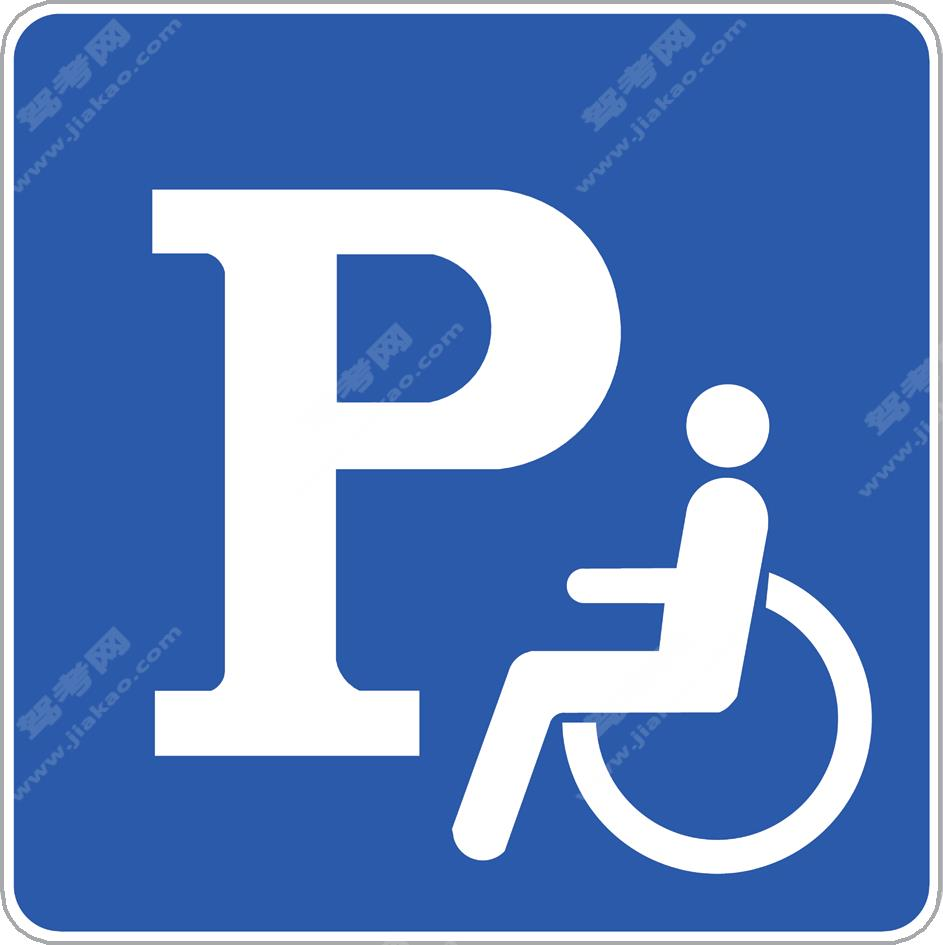残疾人专用停车位（新）

•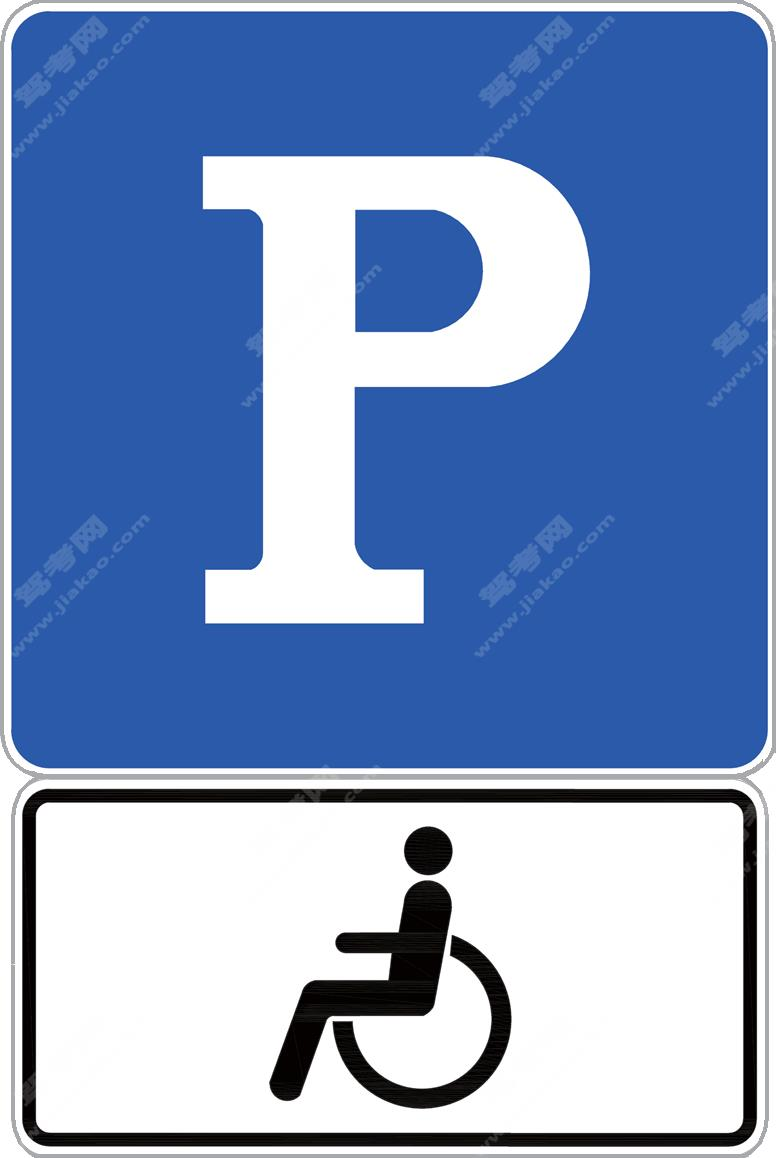残疾人专用停车位（新）

•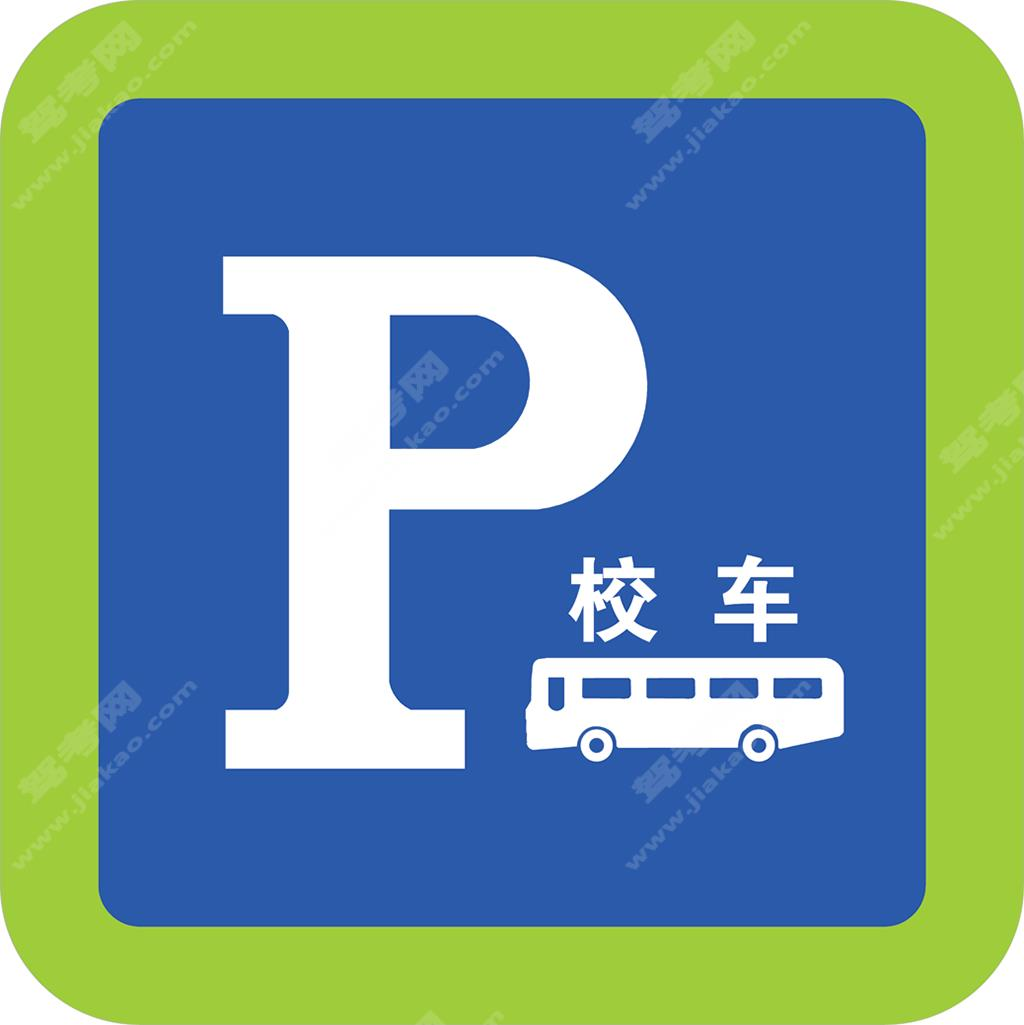校车专用停车位标志、校车停靠站点标志（新）

•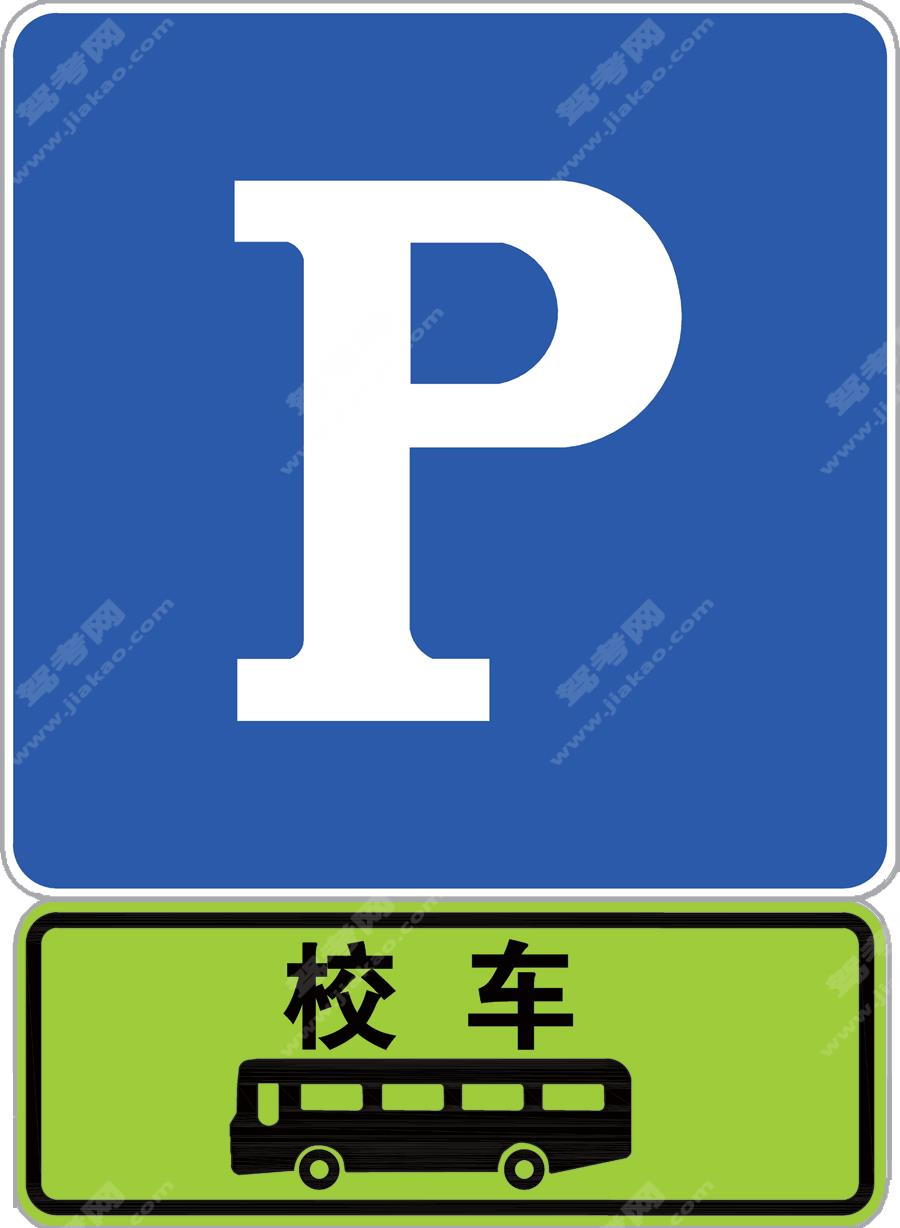校车专用停车位标志、校车停靠站点标志（新）

•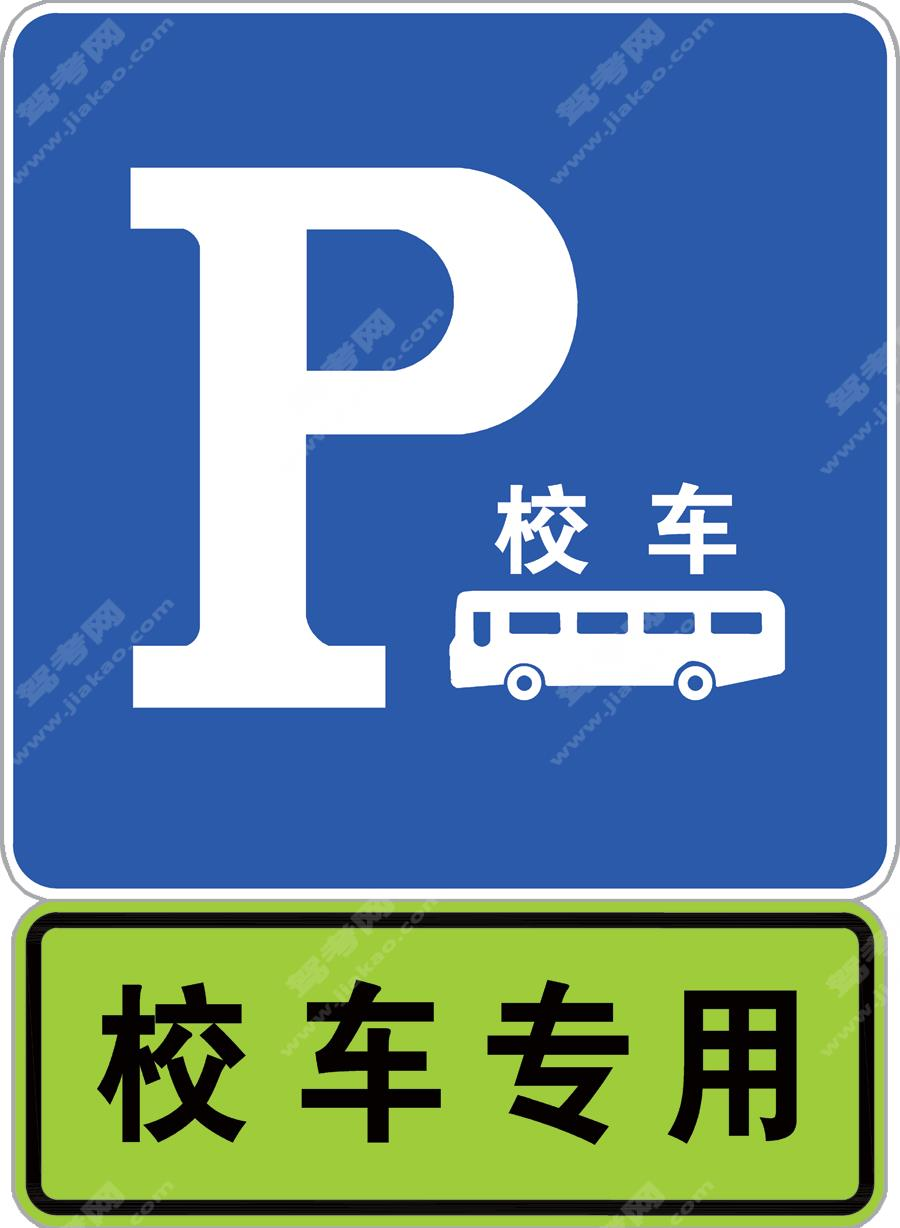校车专用停车位标志、校车停靠站点标志（新）

•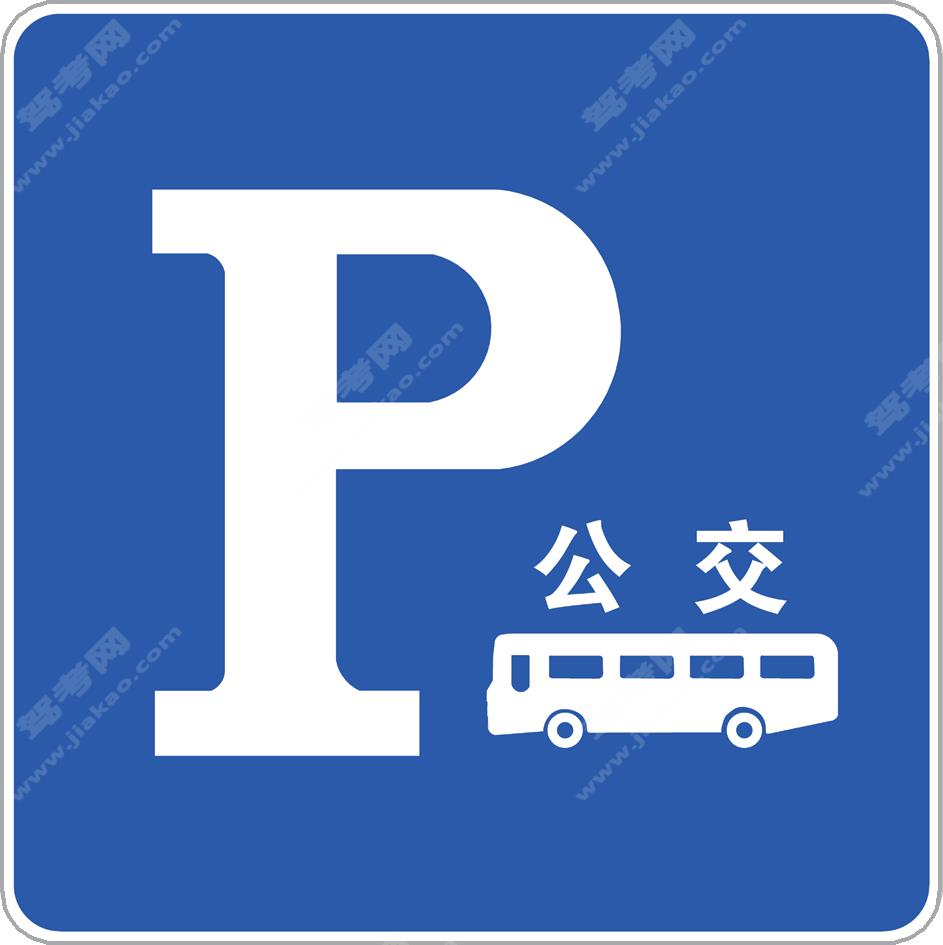公交车专用停车位标志（新）

•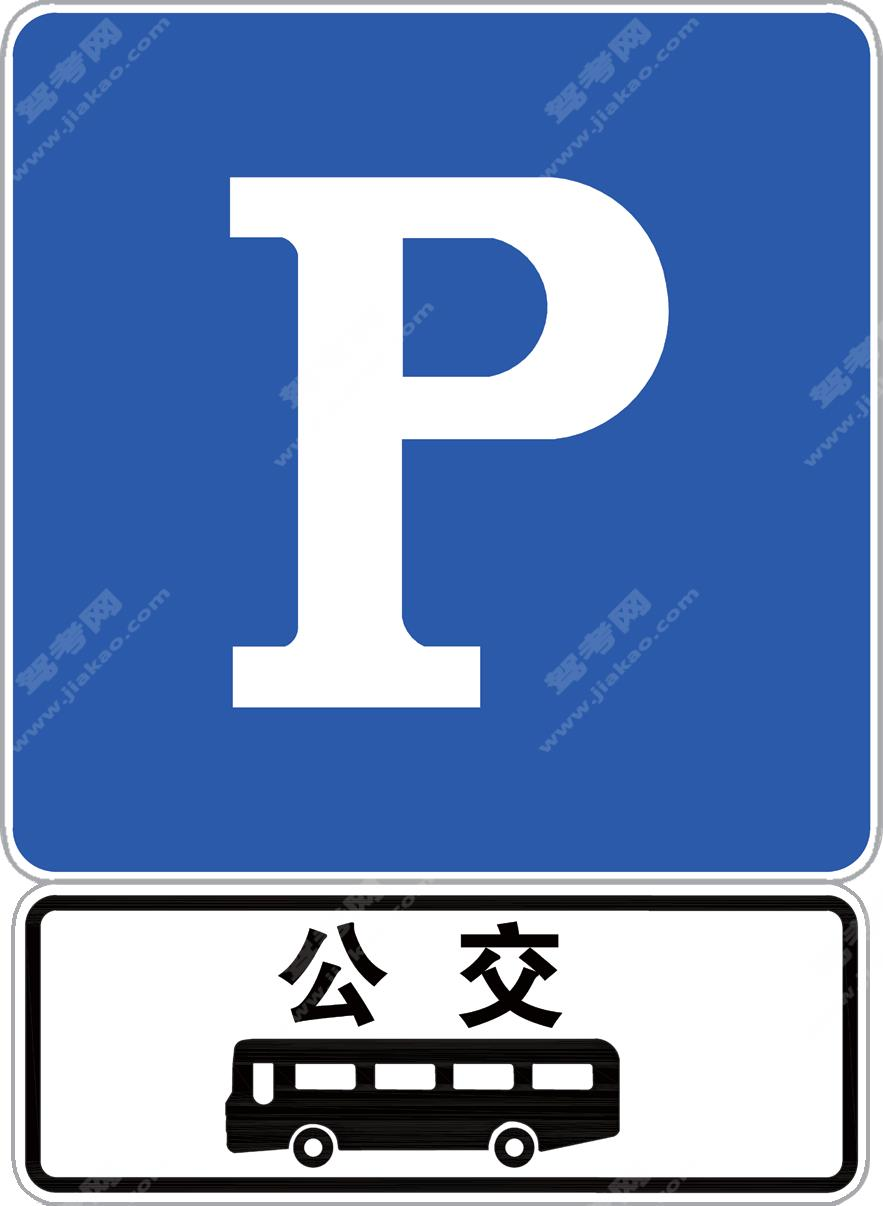公交车专用停车位标志（新）

•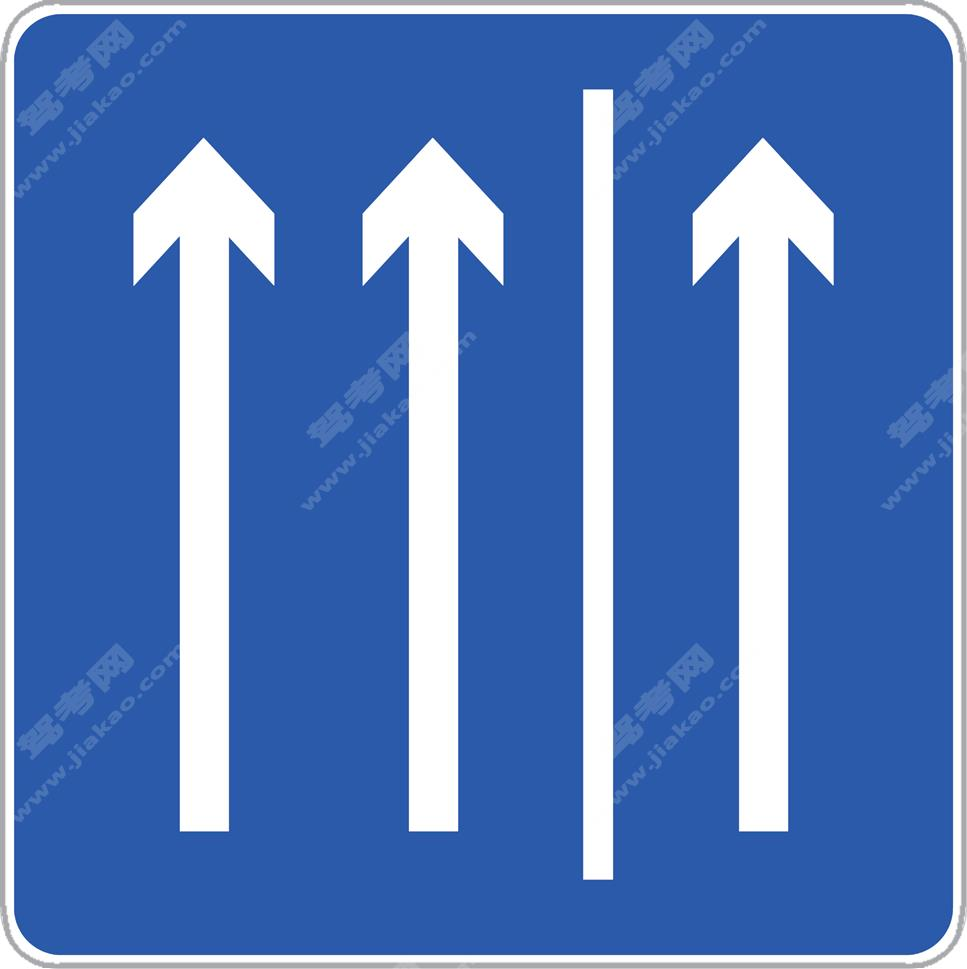硬路肩允许行驶起点

•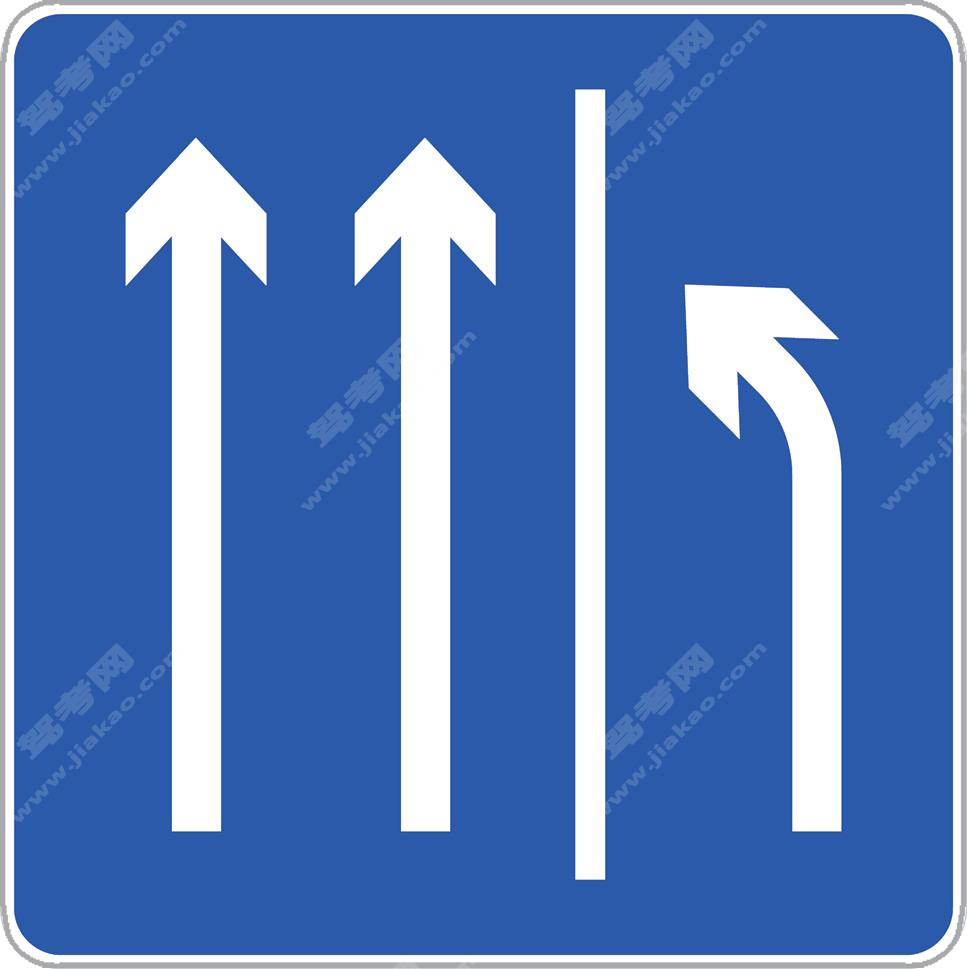硬路肩允许行驶即将结束（新）

•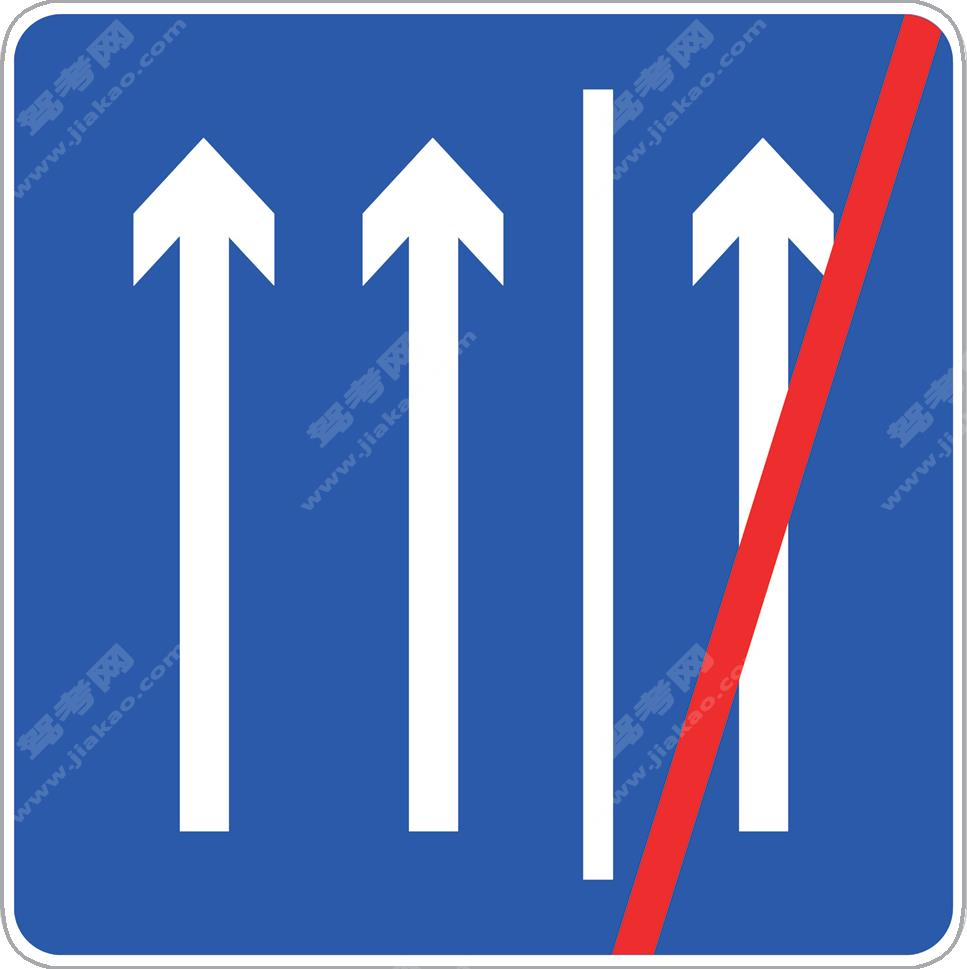硬路肩允许行驶终点（新）

•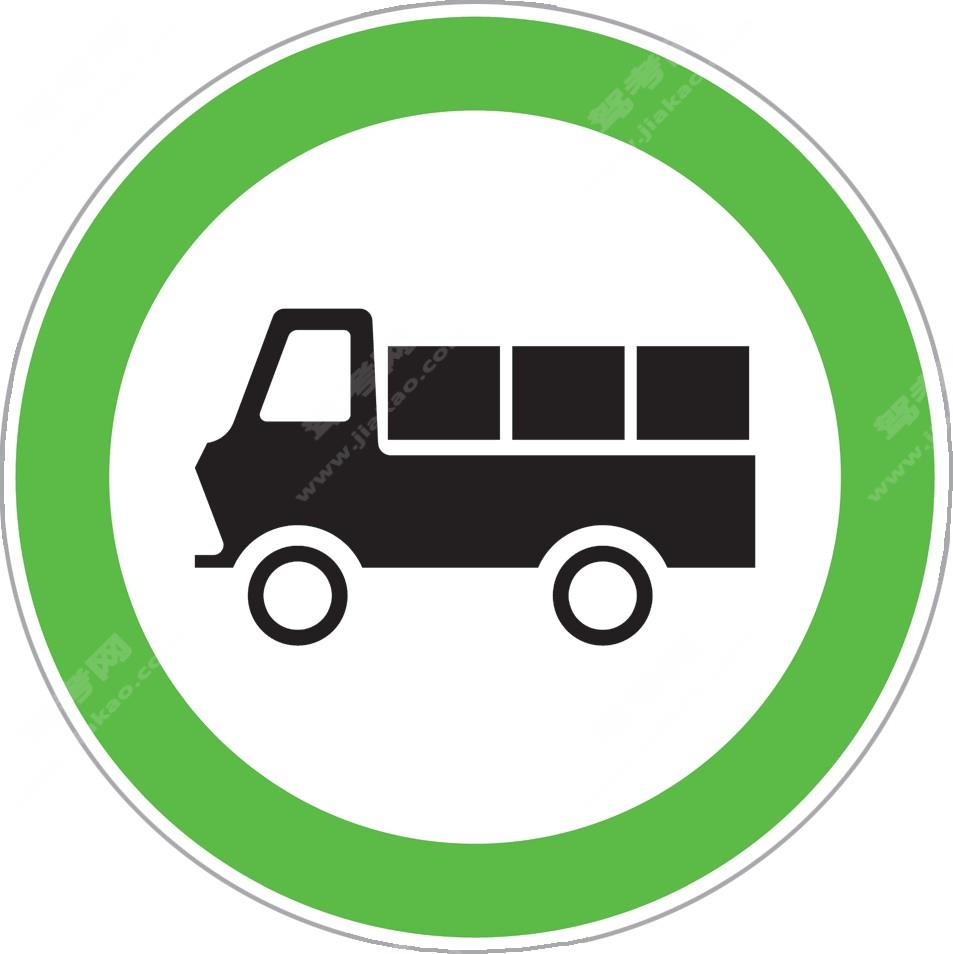货车通行（新）

•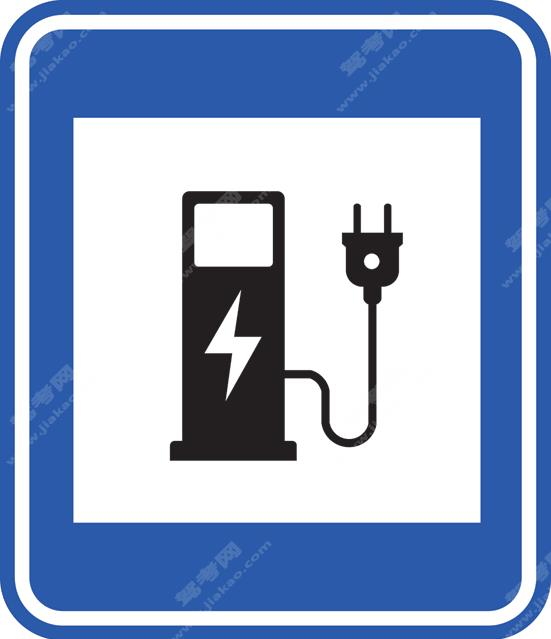电动汽车充电站（新）

•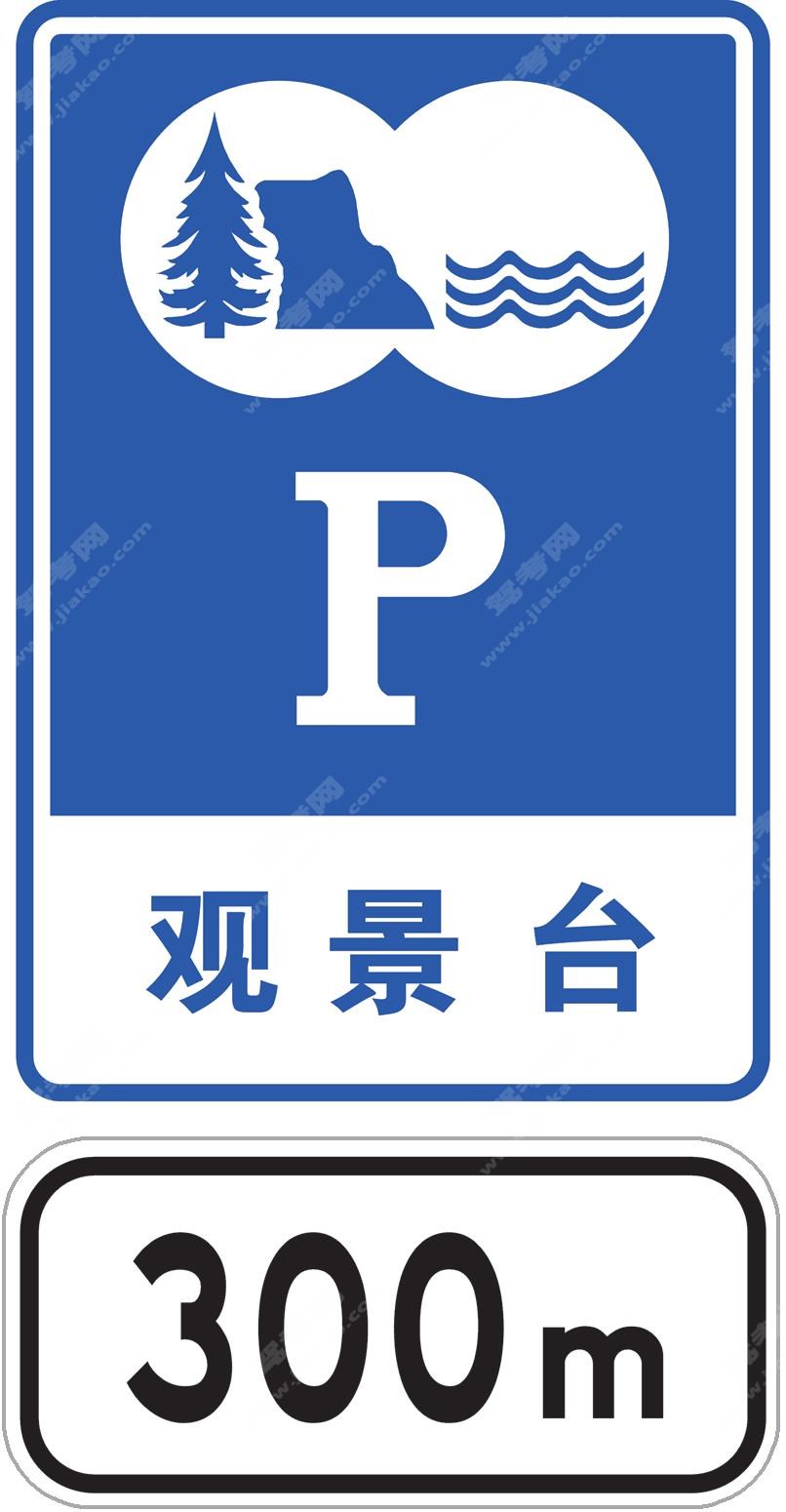观景台标志加辅助标志（新）

•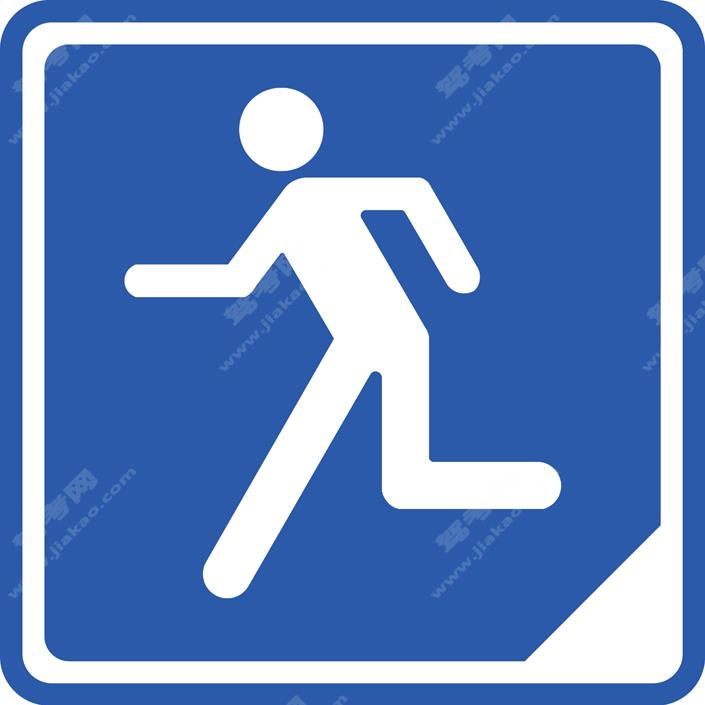应急避难设施（场所）（新）

•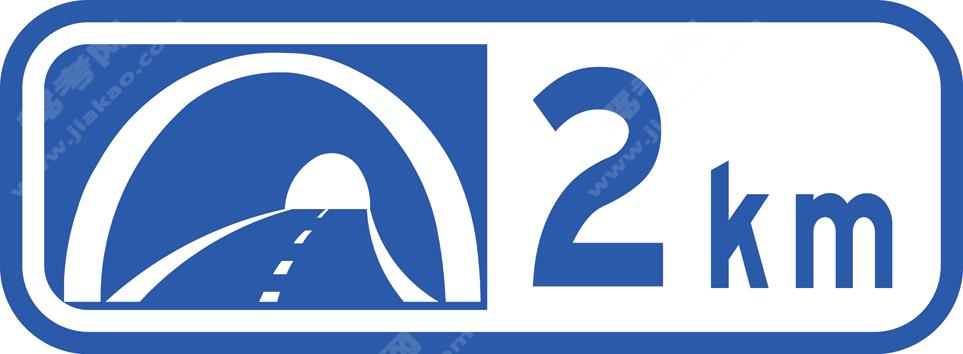隧道出口距离预告（新）

•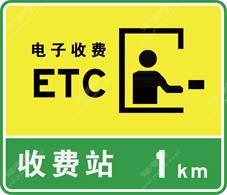设有电子不停车收费(ETC) 车道的收费站预告（新）

•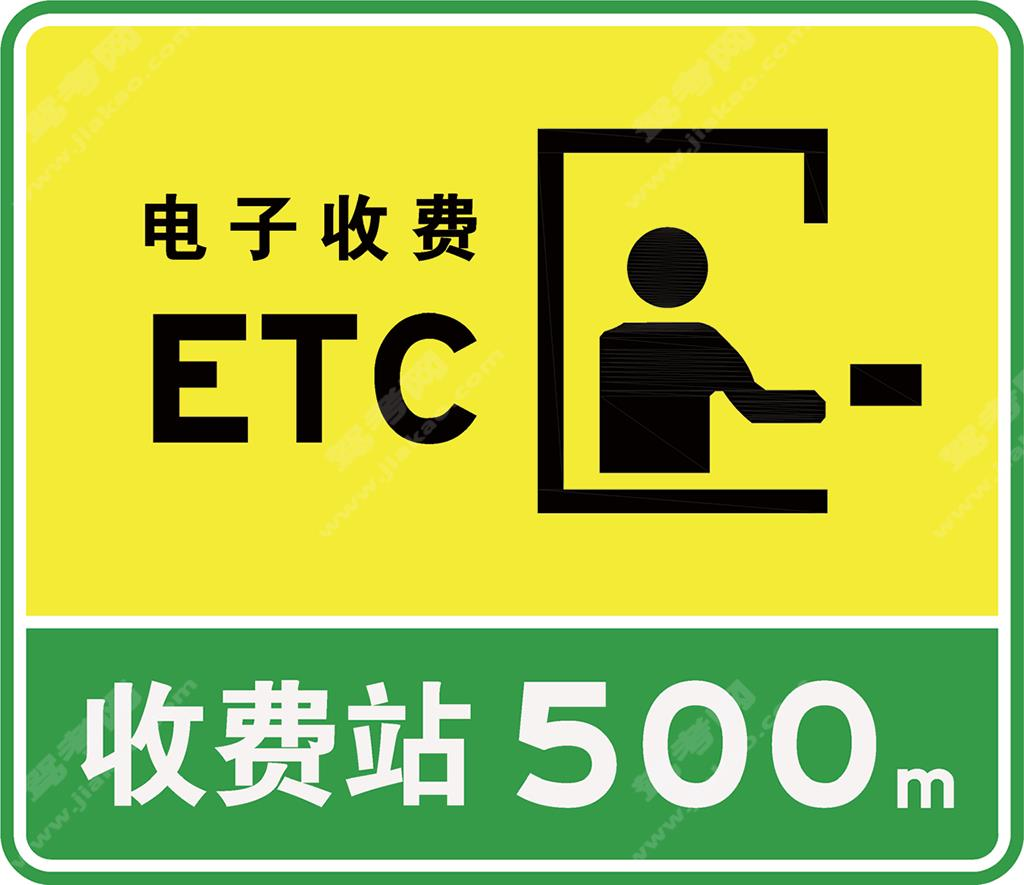设有电子不停车收费(ETC) 车道的收费站预告（新）

•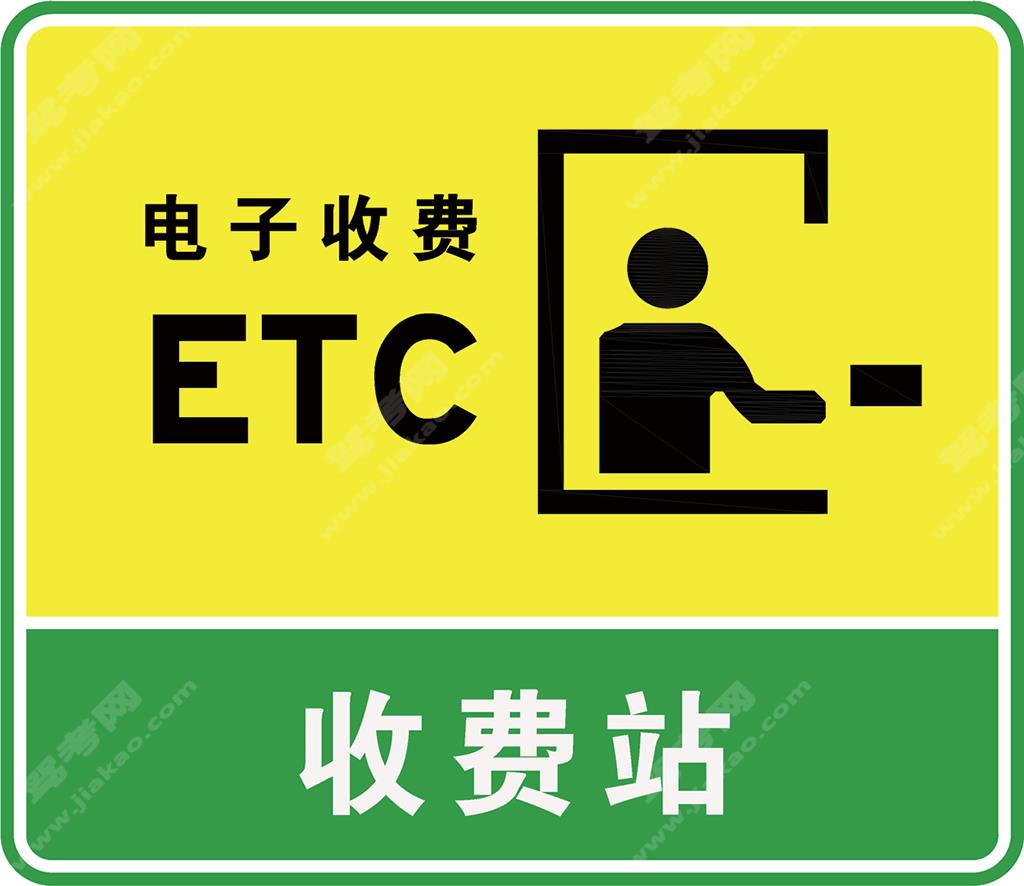设有电子不停车收费(ETC) 车道的收费站预告及收费站（新）

•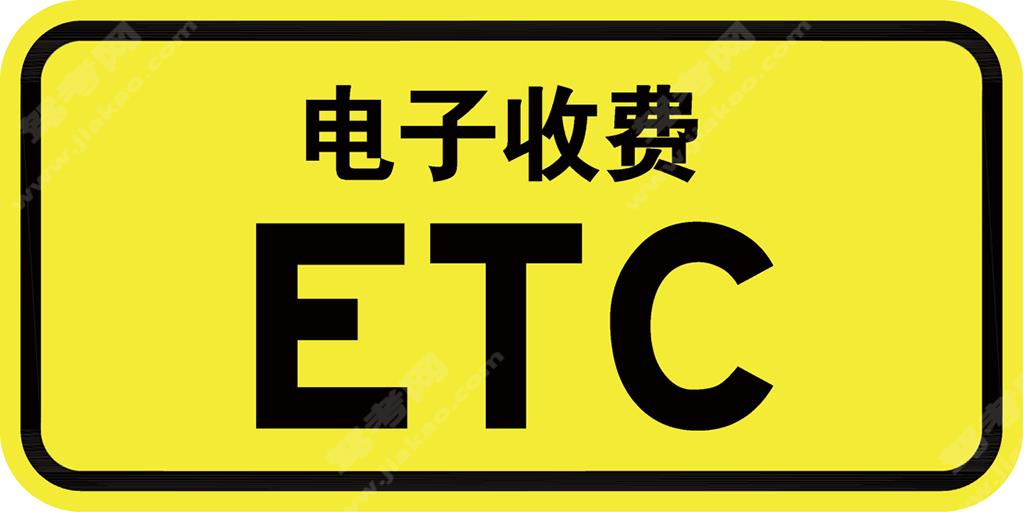电子不停车收费（ETC）车道

•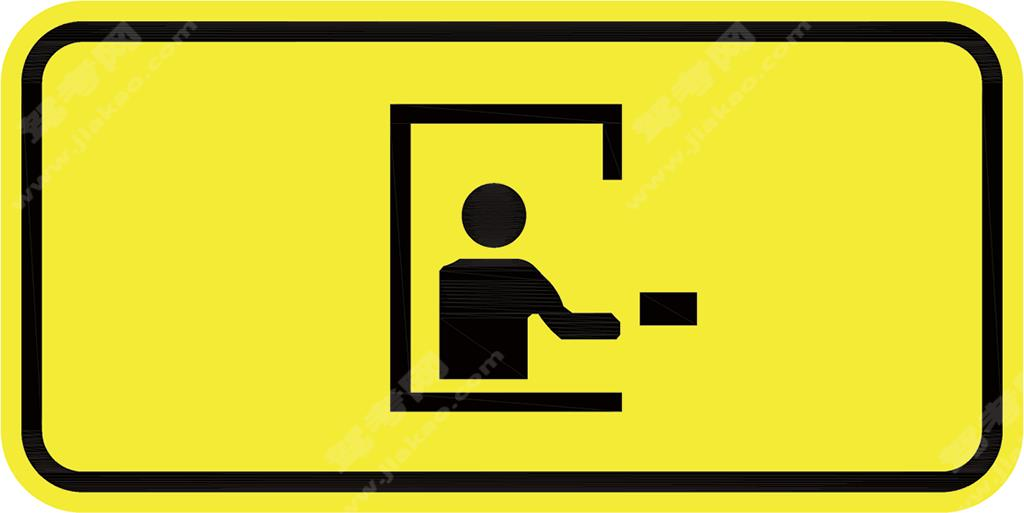人工收费车道（新）

•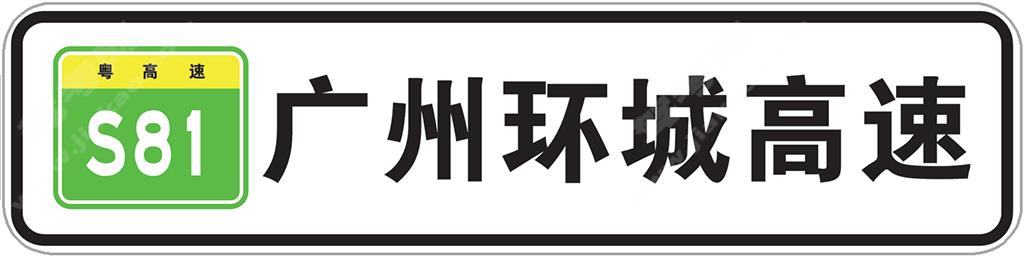高速公路编号信息（新）

•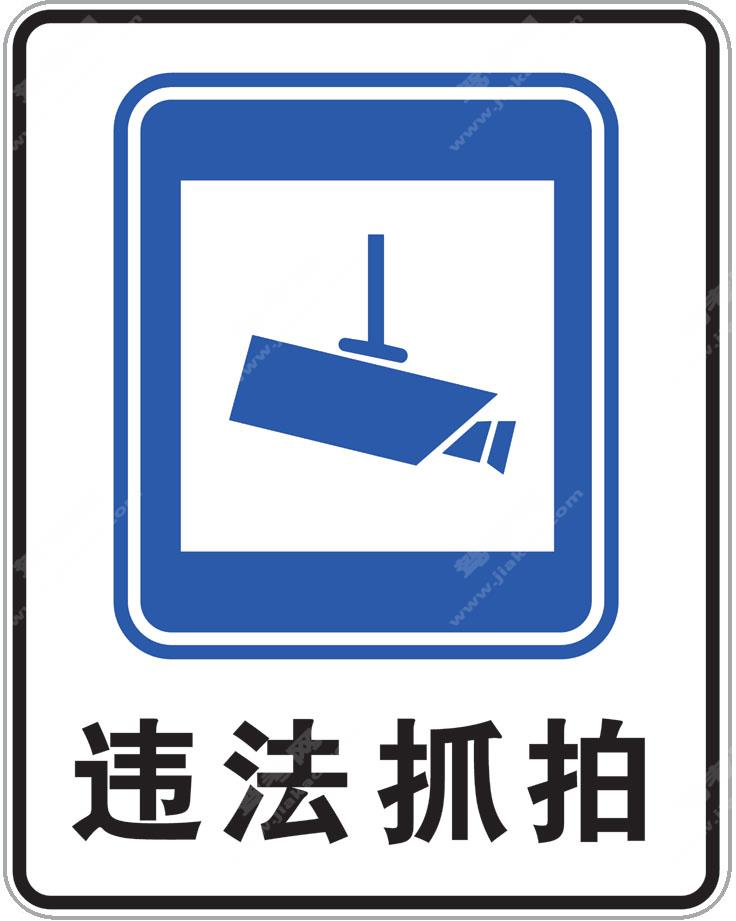交通监控设备信息（新）

•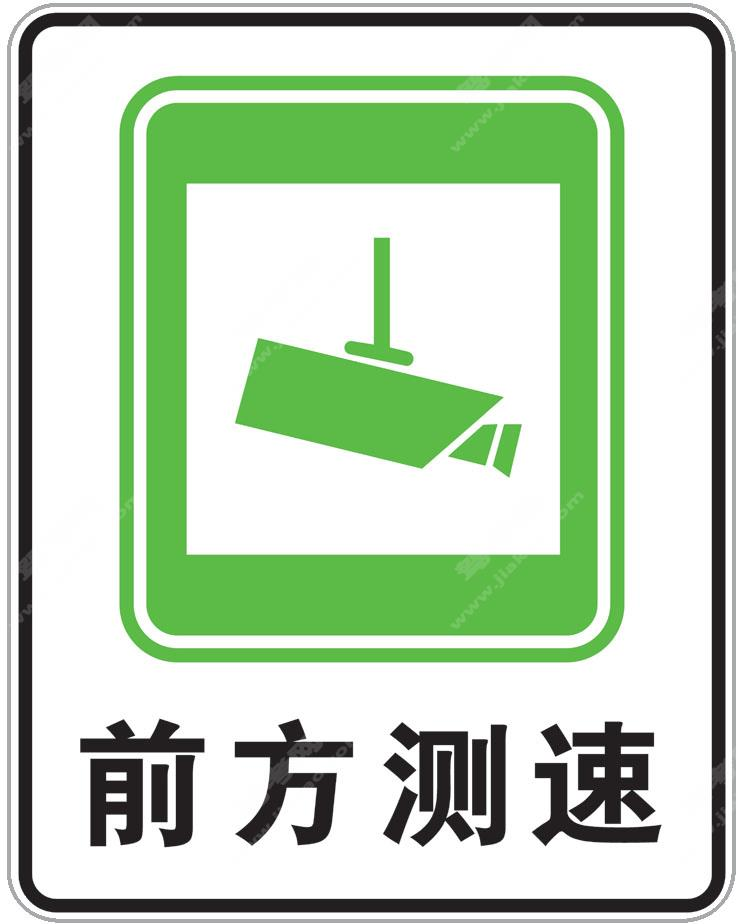交通监控设备信息（新）

•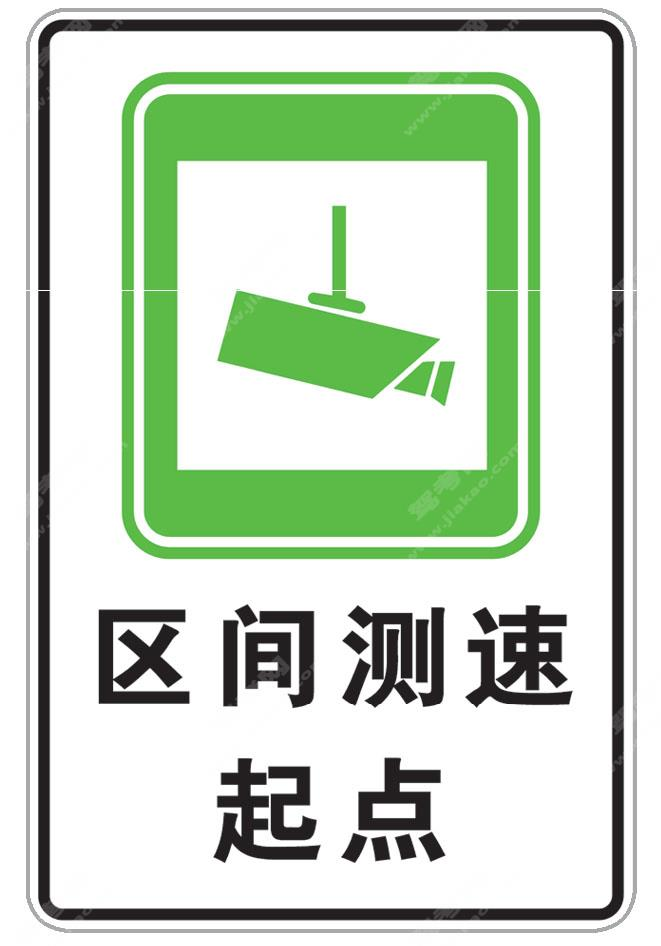区间测速信息（新）

•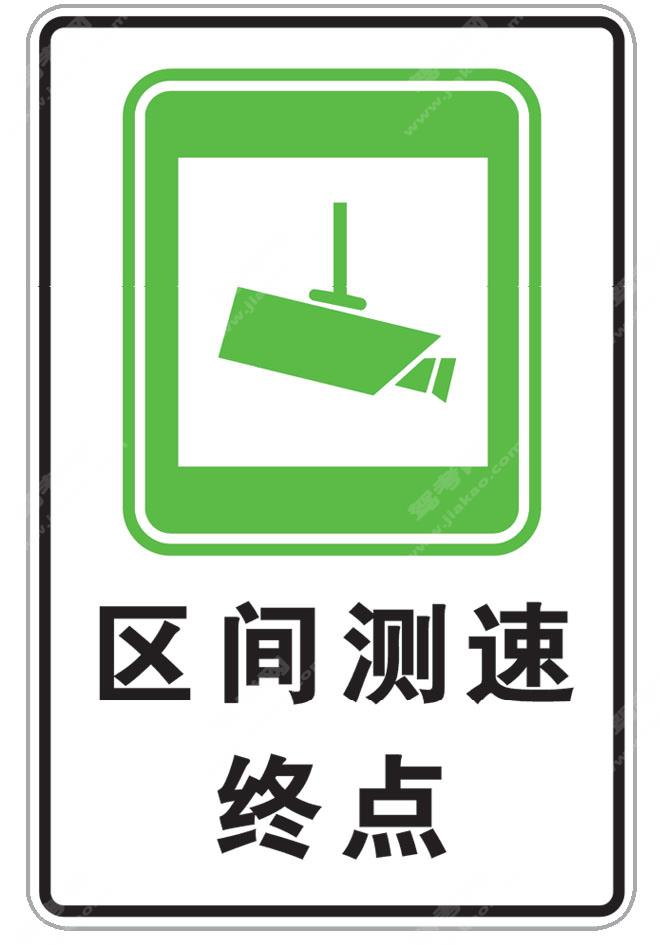区间测速信息（新）

•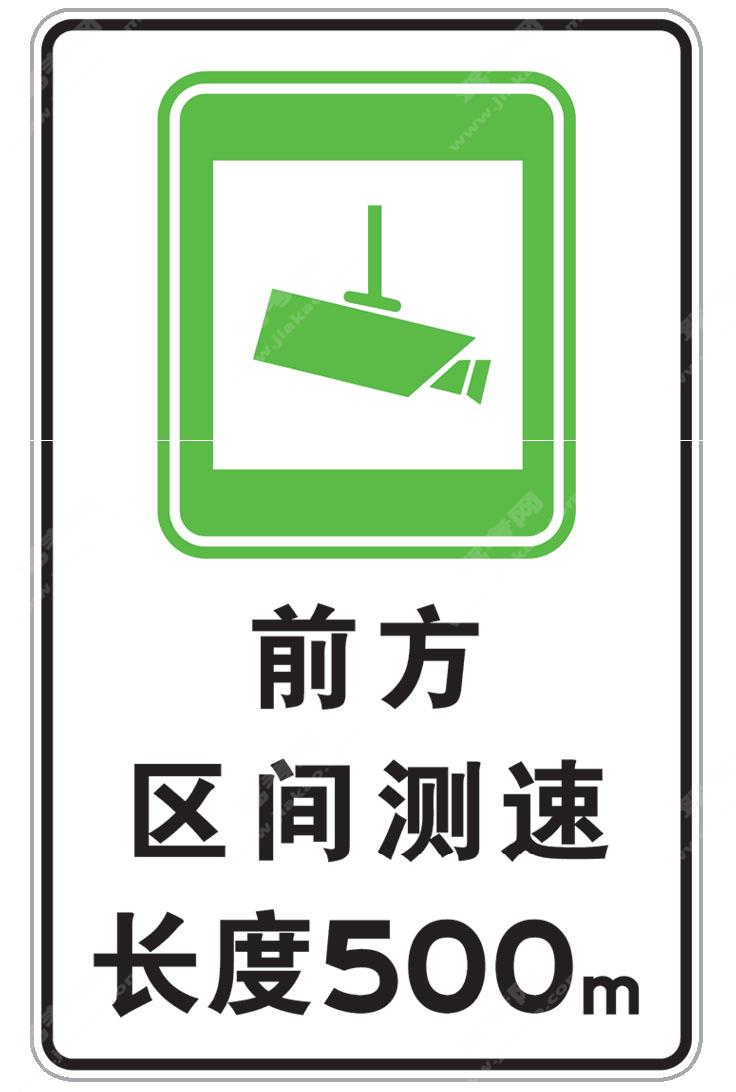区间测速信息（新）

•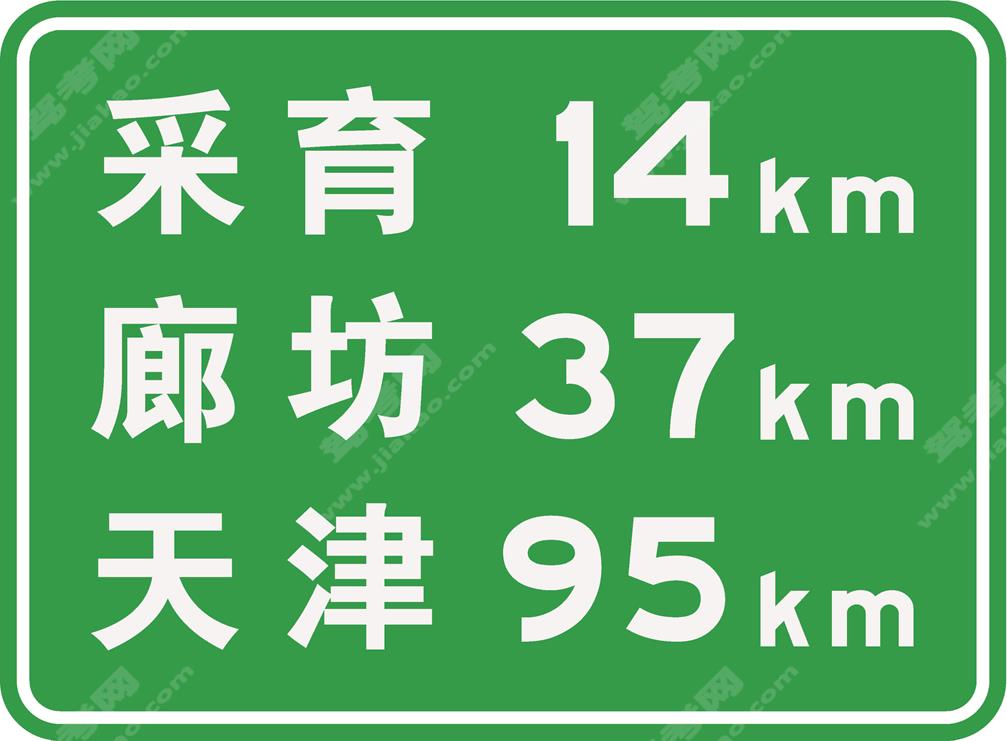地点距离（新）

•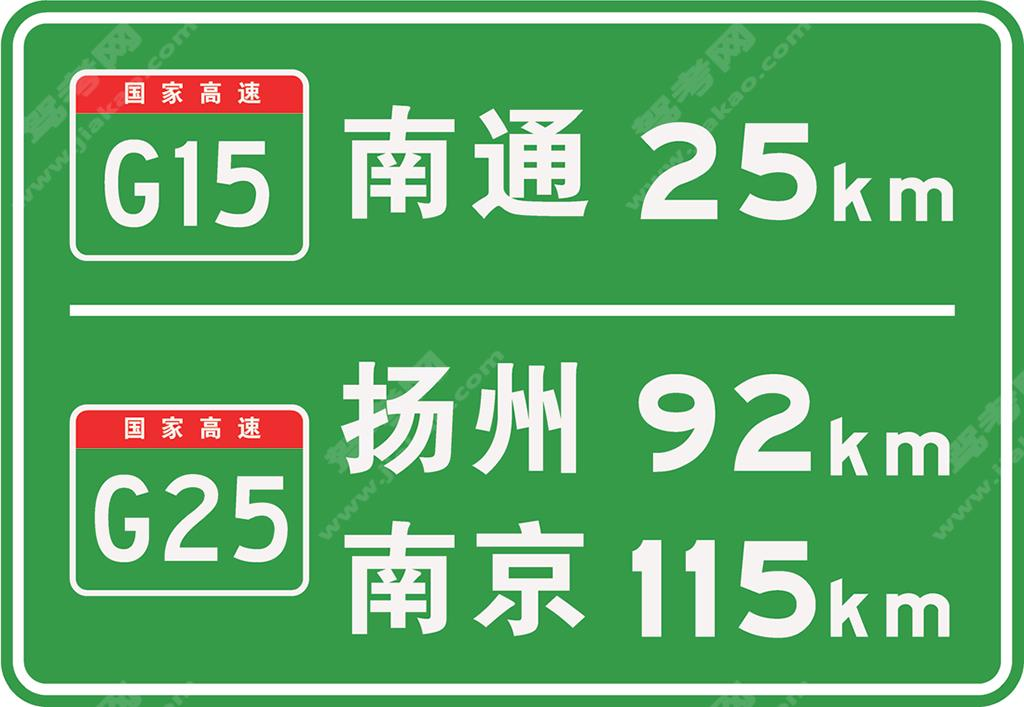城市区域多个出口时的地点距离（新）

•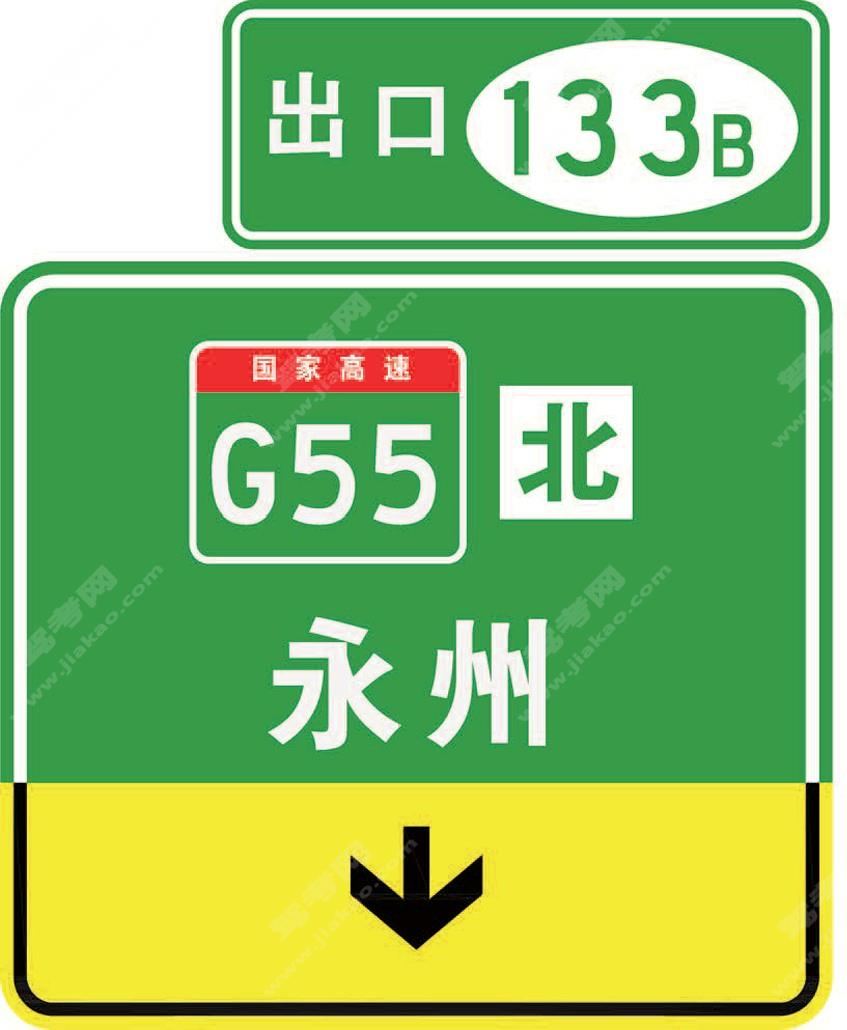直出车道指引（新）

•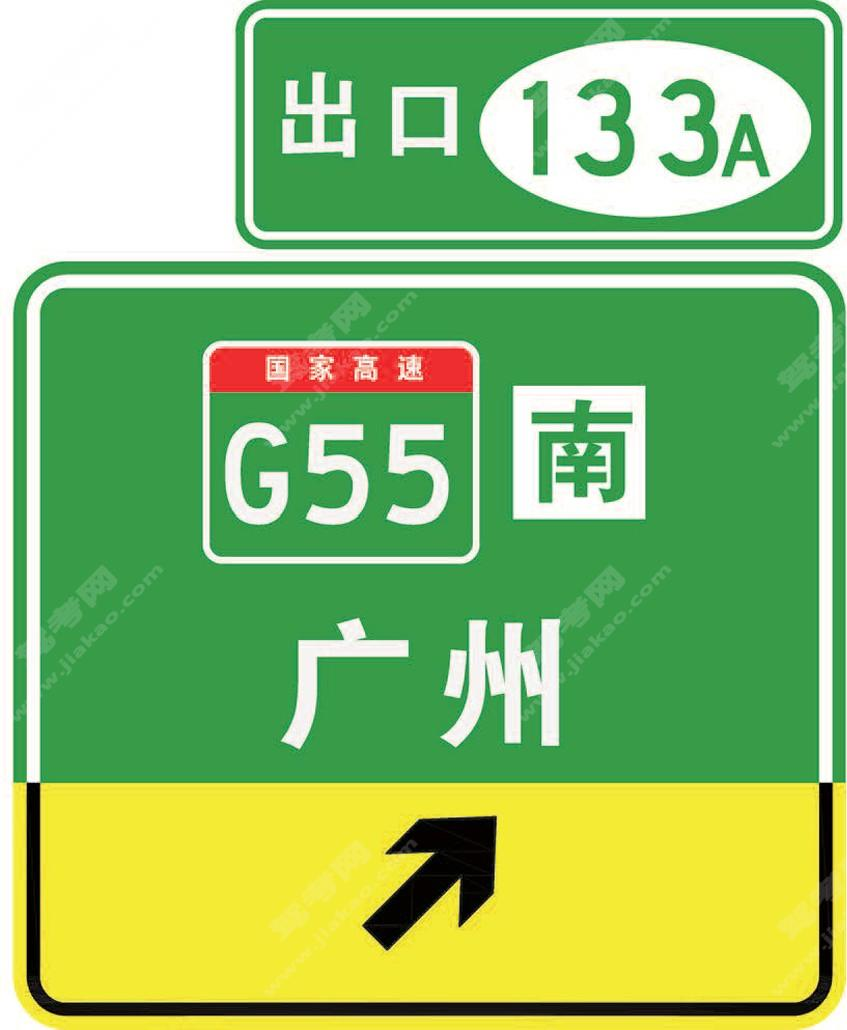直出车道指引（新）

•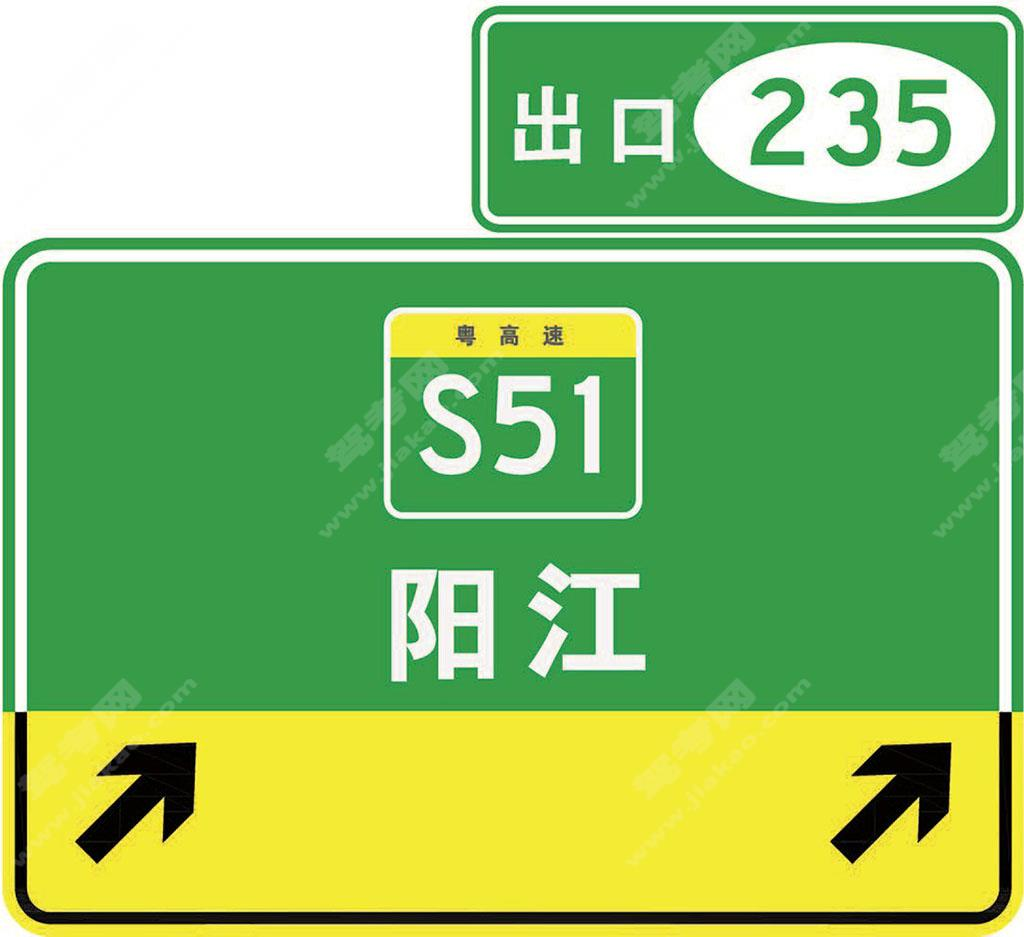直出车道指引（新）

•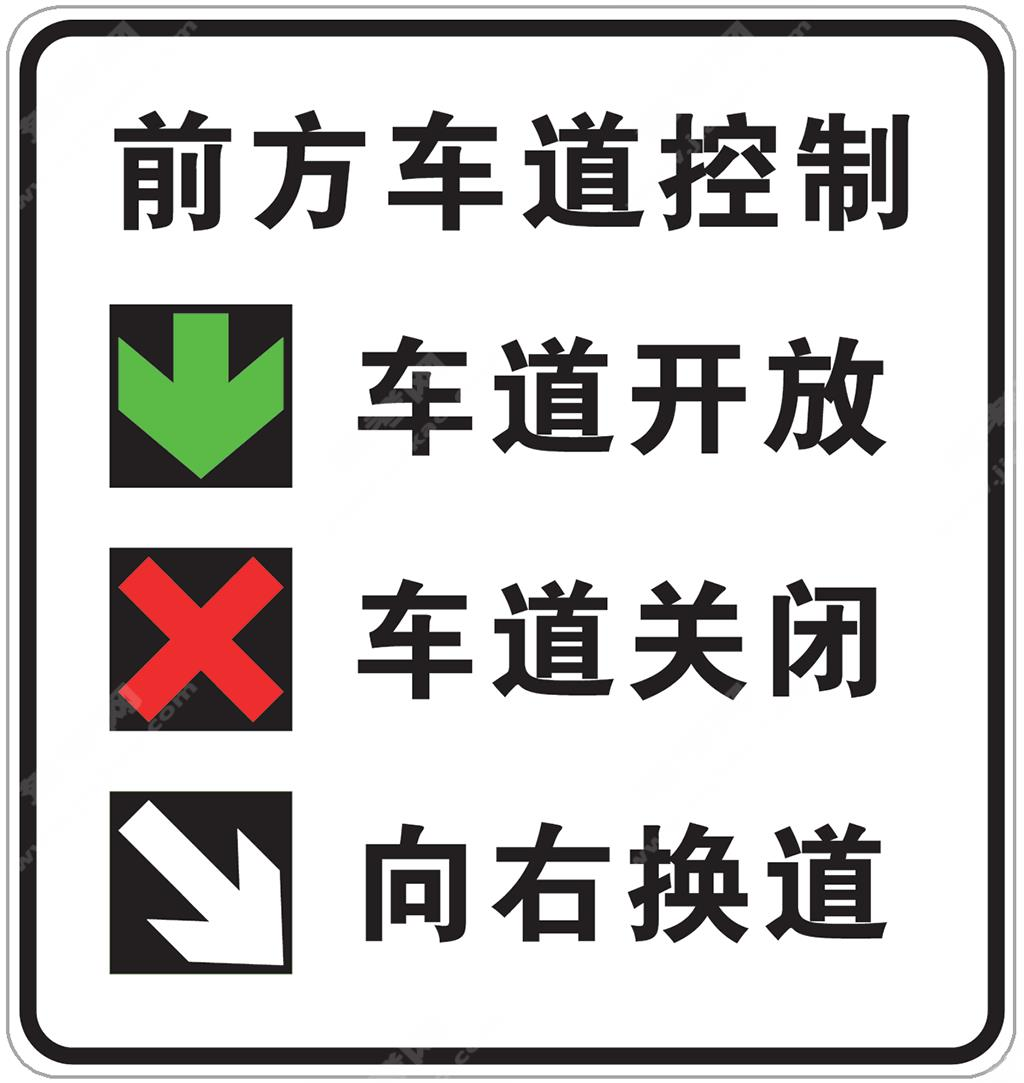前方车道控制（新）

•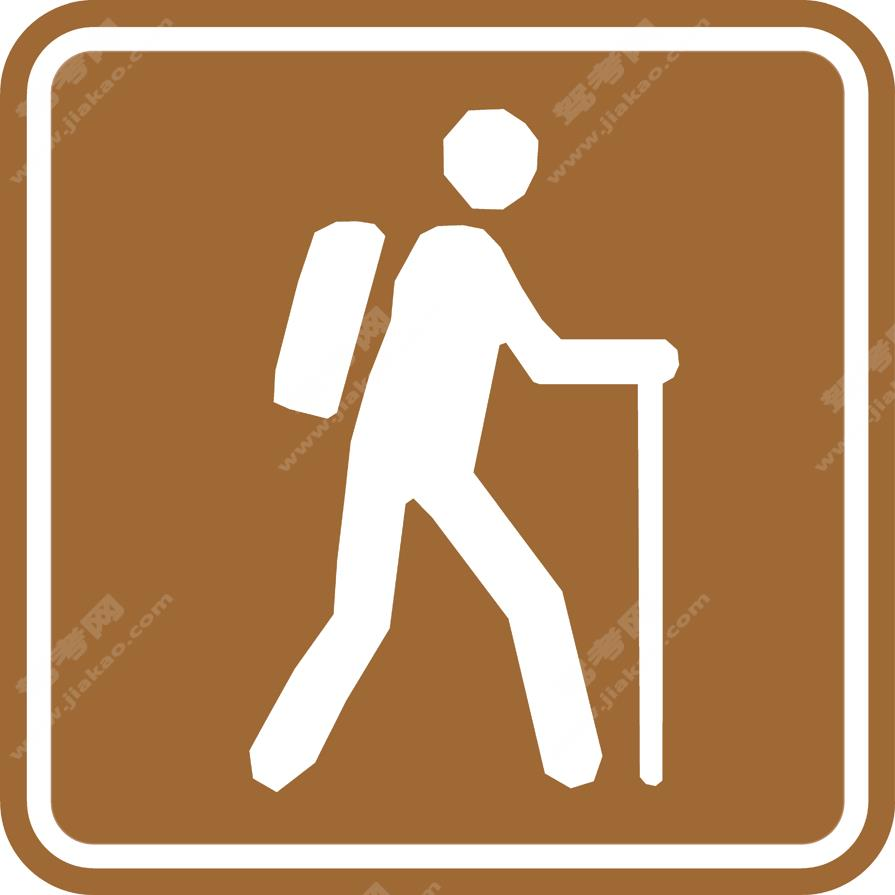徒步（新）

•营火（新）

•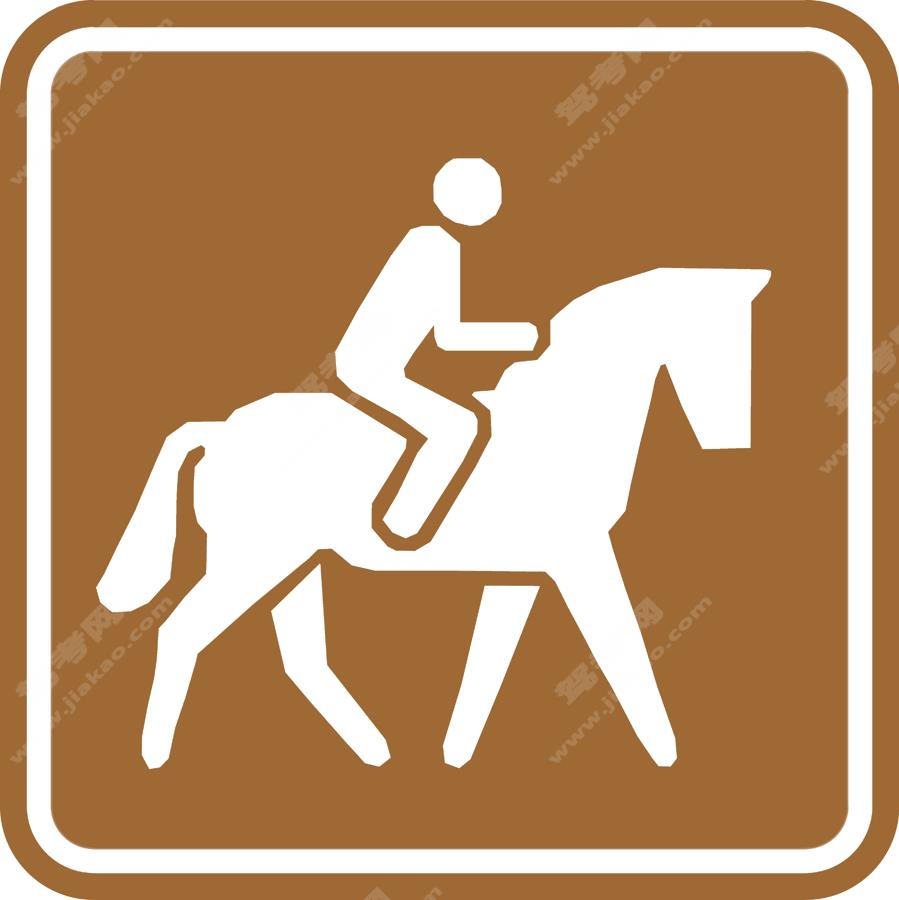骑马（新）

•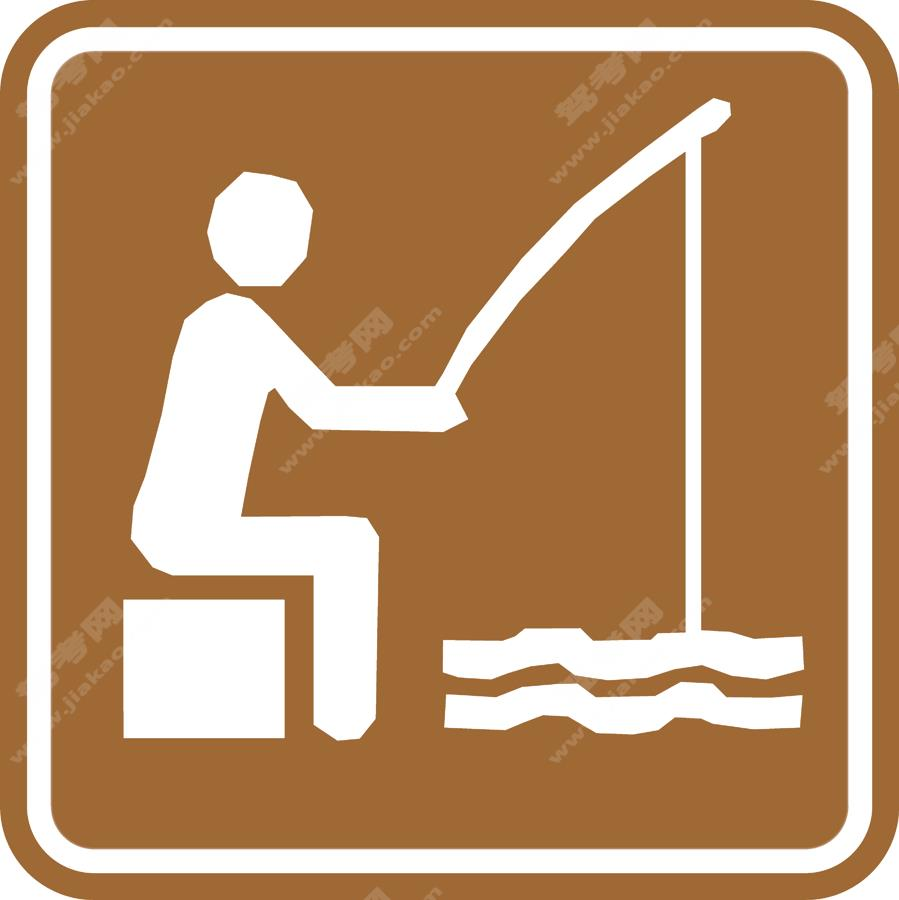钓鱼（新）

•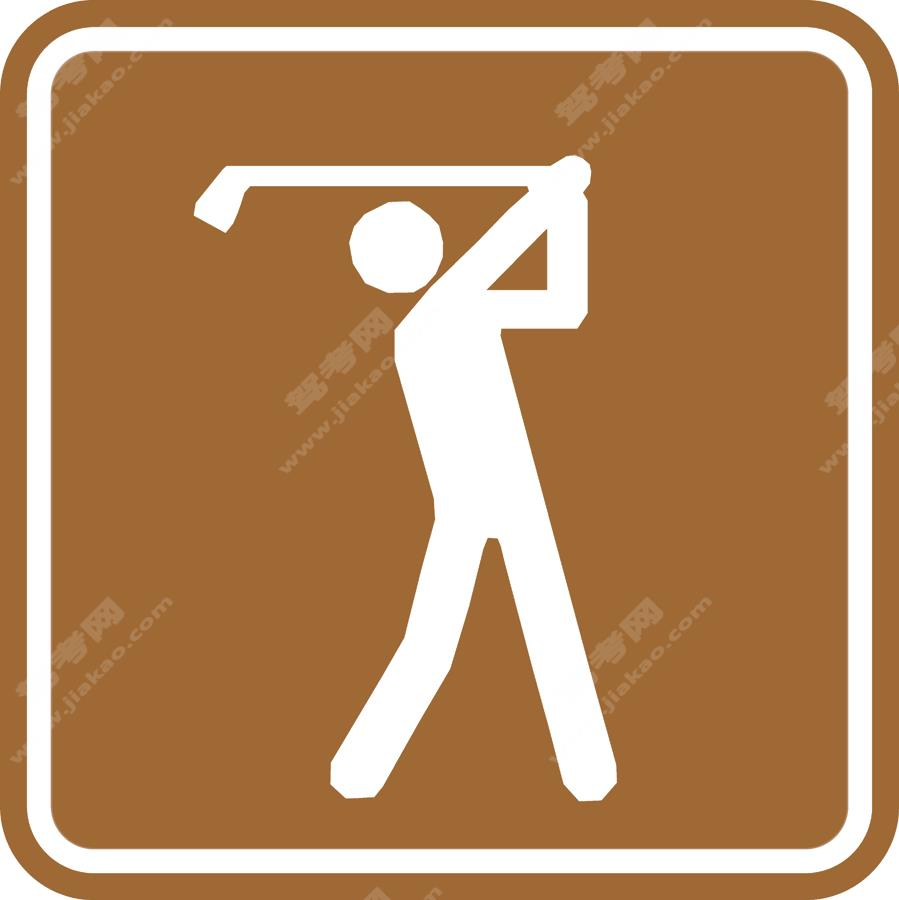高尔夫球（新）

•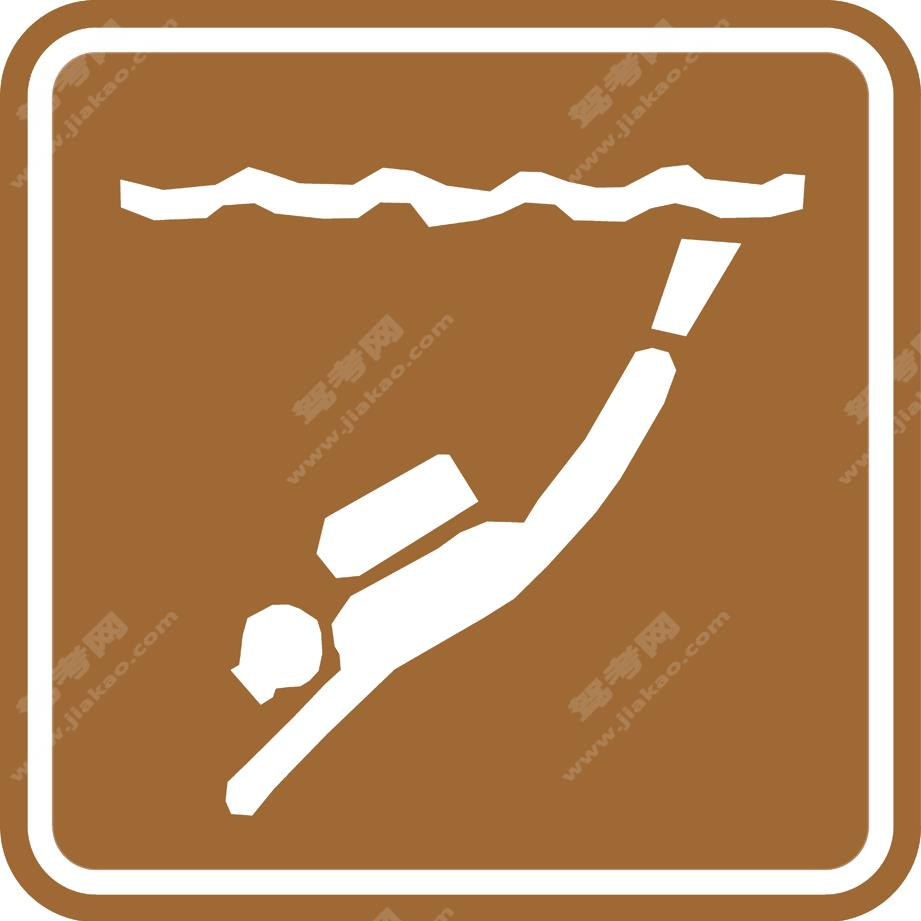潜水（新）

•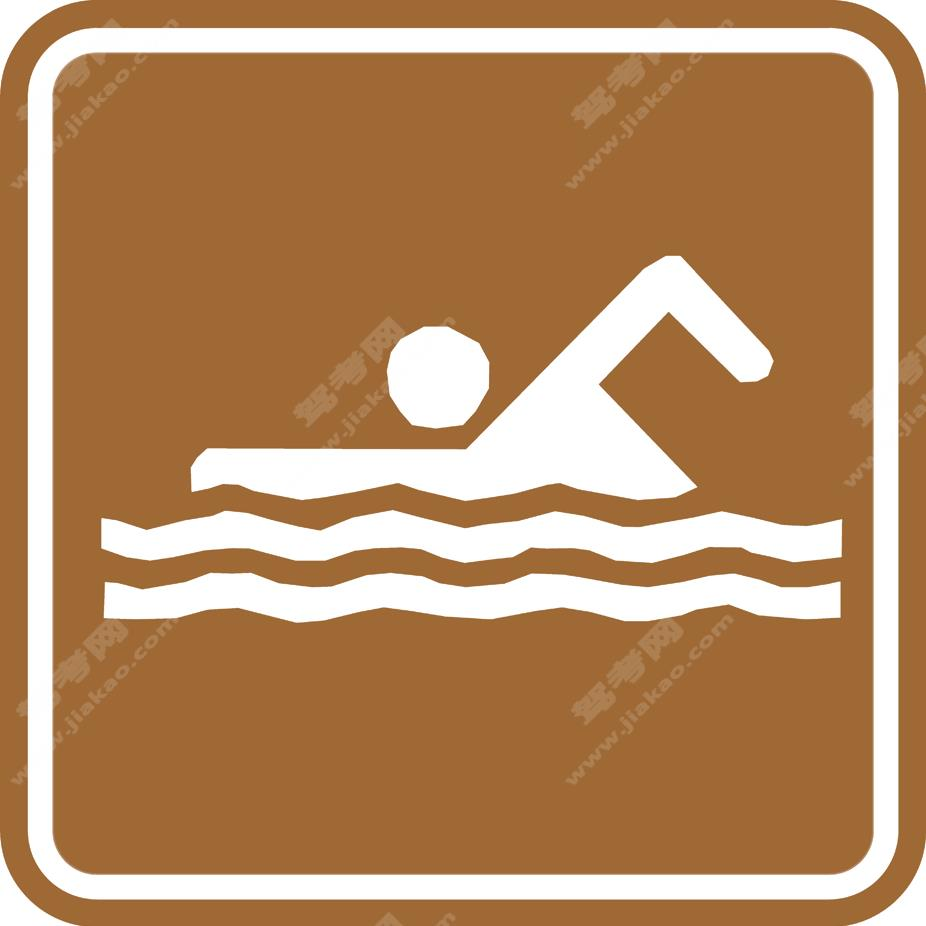游泳（新）

•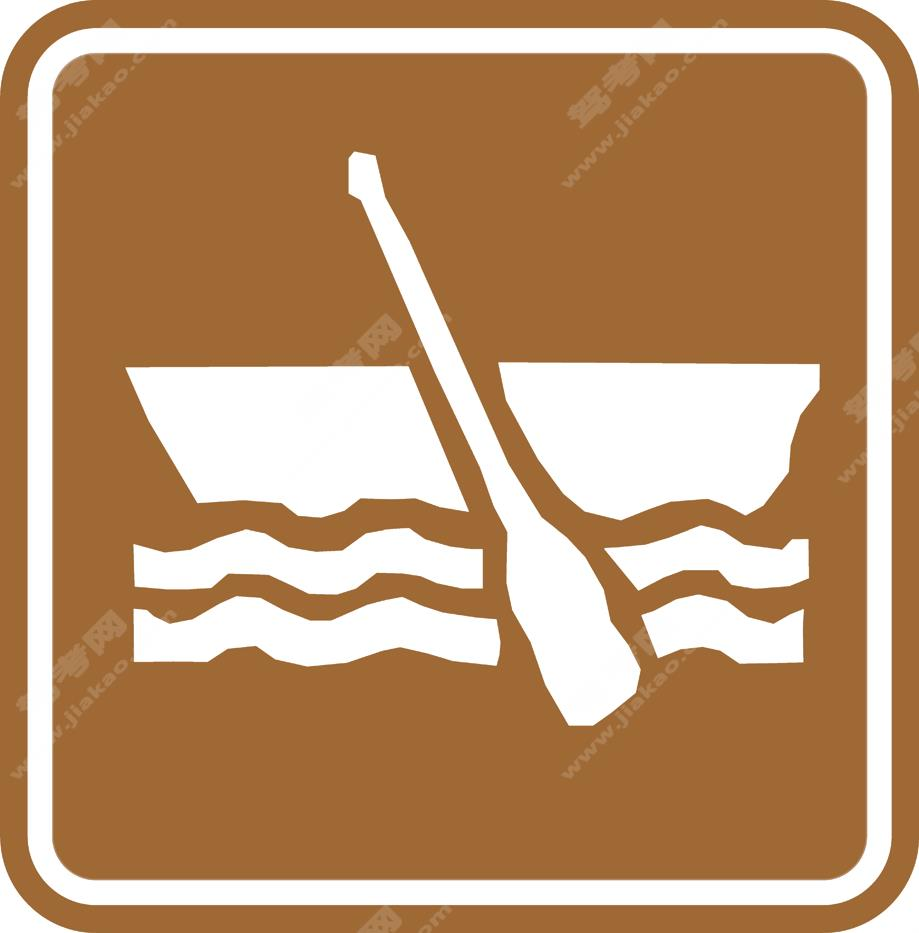划船（新）

•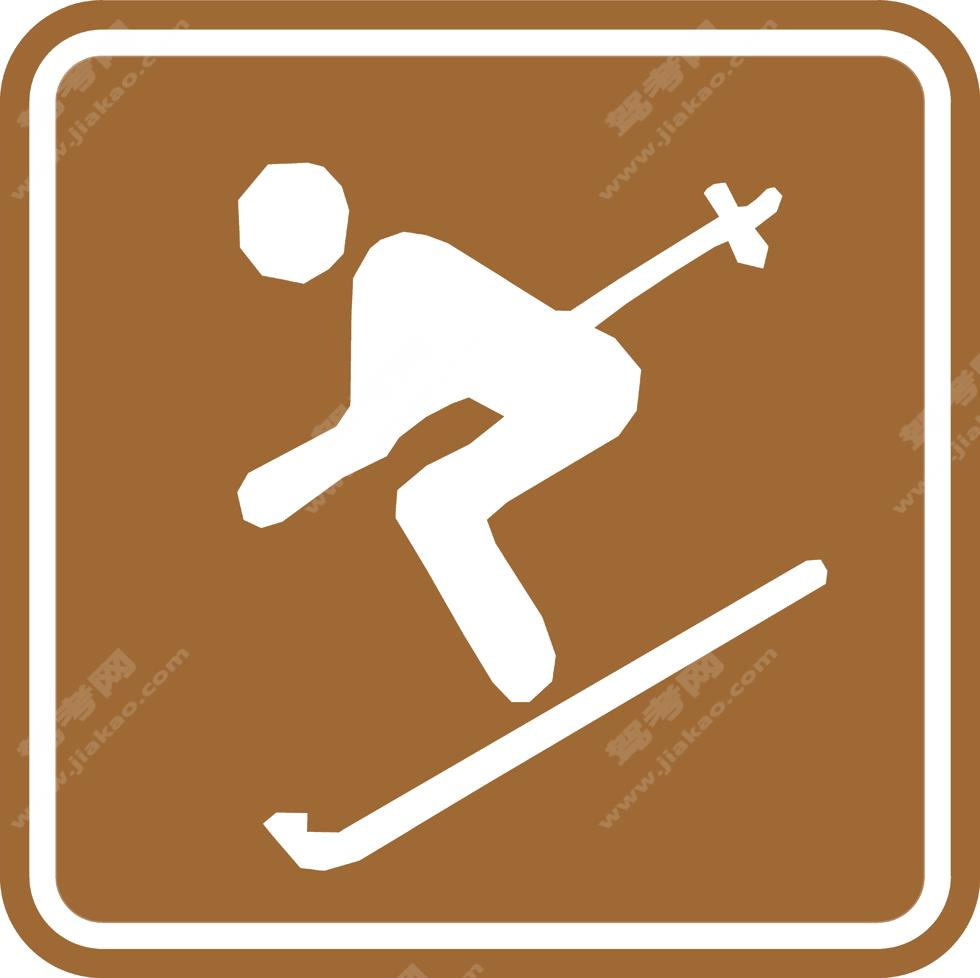滑雪（新）

•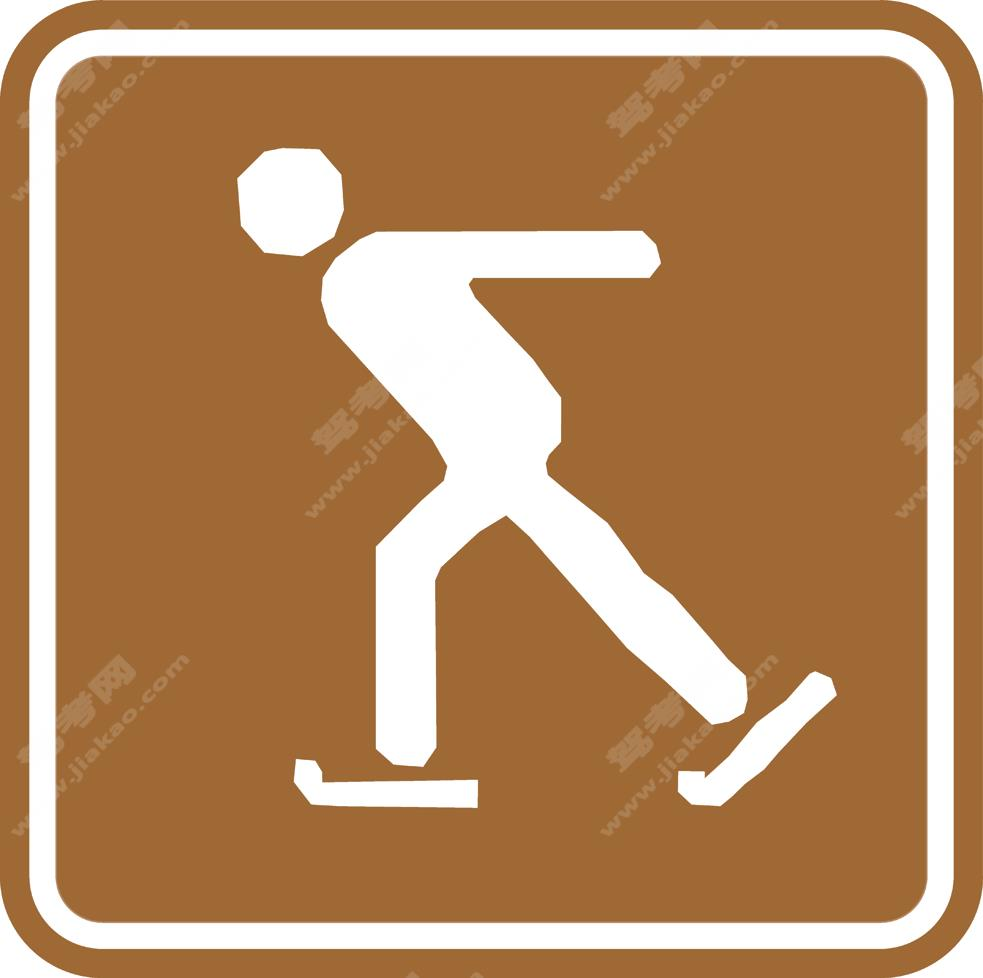滑冰（新）

×